Скачать презентацию Network Security Frank Yeong-Sung Lin Department of Information

0ec5aa9dbe36c716e0aacabd13d039f8.ppt

• Количество слайдов: 78Network Security Frank Yeong-Sung Lin Department of Information Management National Taiwan University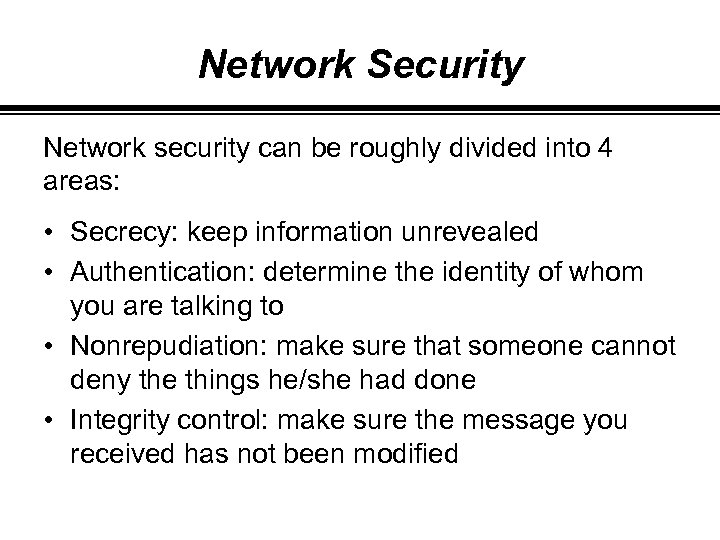Network Security Network security can be roughly divided into 4 areas: • Secrecy: keep information unrevealed • Authentication: determine the identity of whom you are talking to • Nonrepudiation: make sure that someone cannot deny the things he/she had done • Integrity control: make sure the message you received has not been modified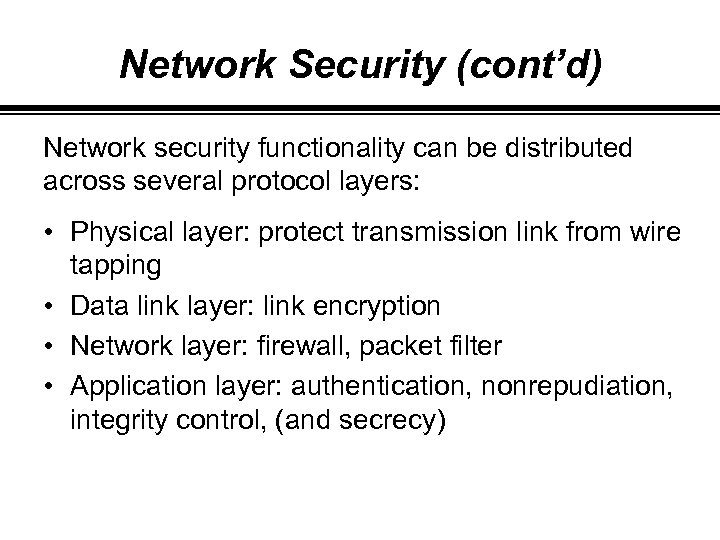Network Security (cont’d) Network security functionality can be distributed across several protocol layers: • Physical layer: protect transmission link from wire tapping • Data link layer: link encryption • Network layer: firewall, packet filter • Application layer: authentication, nonrepudiation, integrity control, (and secrecy)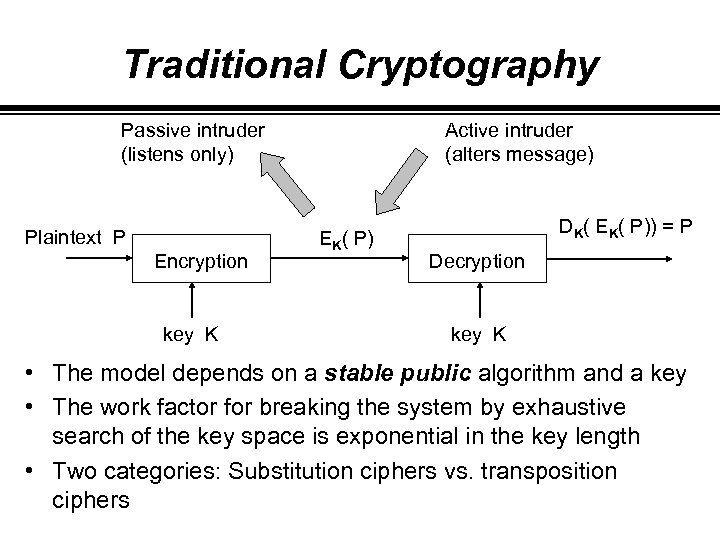Traditional Cryptography Passive intruder (listens only) Plaintext P Encryption key K Active intruder (alters message) EK( P) DK( EK( P)) = P Decryption key K • The model depends on a stable public algorithm and a key • The work factor for breaking the system by exhaustive search of the key space is exponential in the key length • Two categories: Substitution ciphers vs. transposition ciphers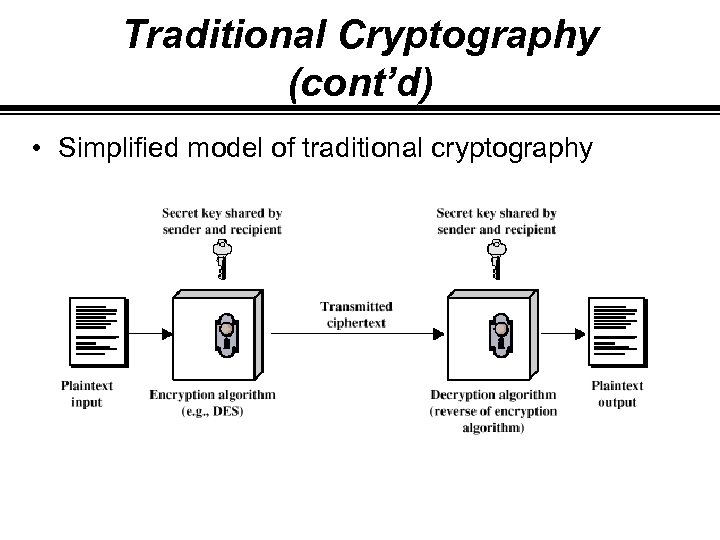Traditional Cryptography (cont’d) • Simplified model of traditional cryptography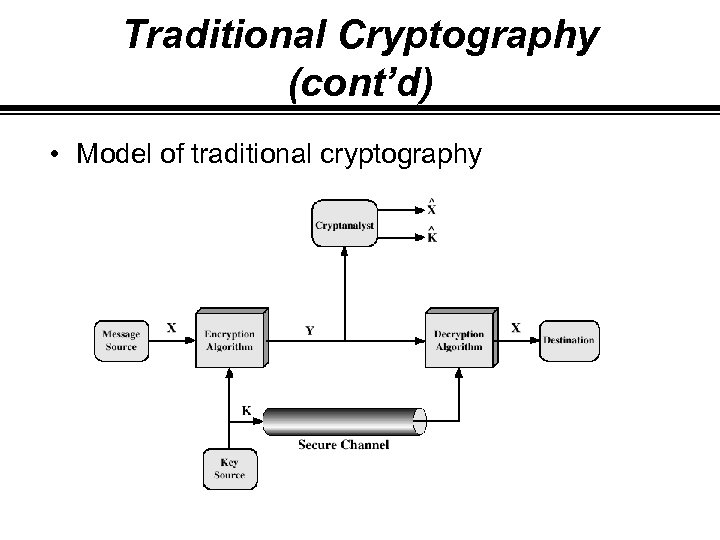Traditional Cryptography (cont’d) • Model of traditional cryptography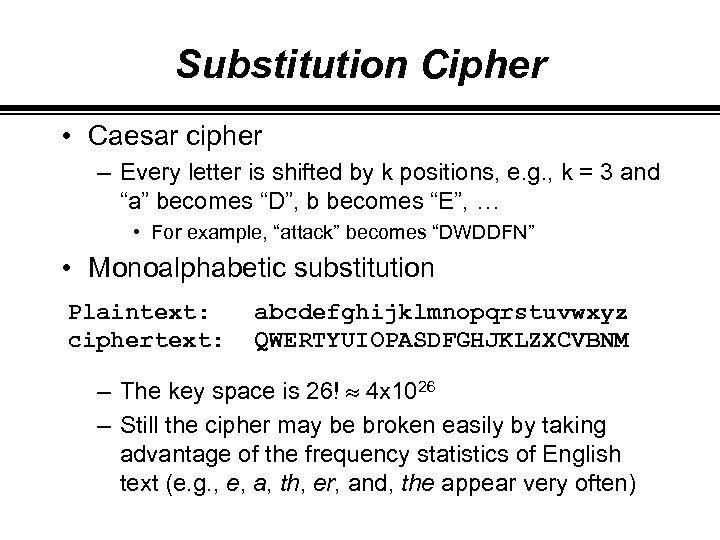Substitution Cipher • Caesar cipher – Every letter is shifted by k positions, e. g. , k = 3 and “a” becomes “D”, b becomes “E”, … • For example, “attack” becomes “DWDDFN” • Monoalphabetic substitution Plaintext: ciphertext: abcdefghijklmnopqrstuvwxyz QWERTYUIOPASDFGHJKLZXCVBNM – The key space is 26! » 4 x 1026 – Still the cipher may be broken easily by taking advantage of the frequency statistics of English text (e. g. , e, a, th, er, and, the appear very often)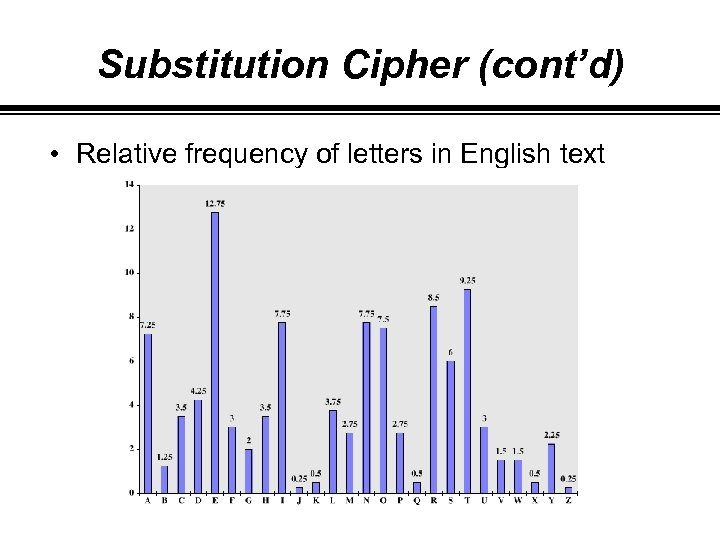Substitution Cipher (cont’d) • Relative frequency of letters in English text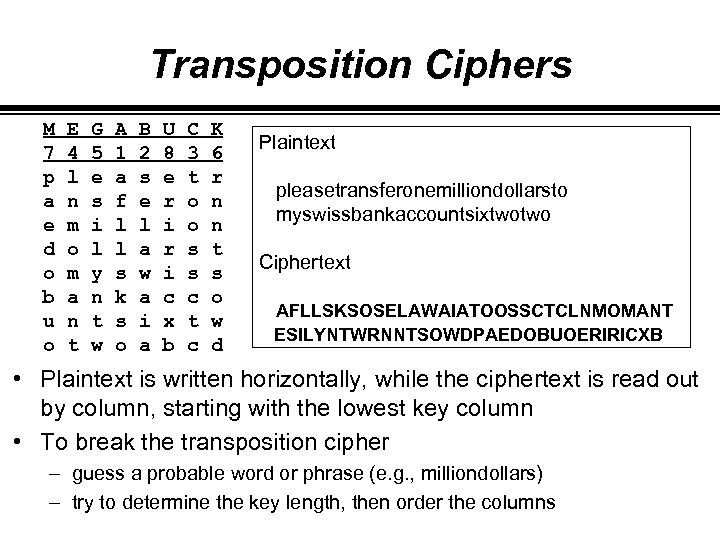Transposition Ciphers M 7 p a e d o b u o E 4 l n m o m a n t G 5 e s i l y n t w A 1 a f l l s k s o B 2 s e l a w a i a U 8 e r i c x b C 3 t o o s s c t c K 6 r n n t s o w d Plaintext pleasetransferonemilliondollarsto myswissbankaccountsixtwotwo Ciphertext AFLLSKSOSELAWAIATOOSSCTCLNMOMANT ESILYNTWRNNTSOWDPAEDOBUOERIRICXB • Plaintext is written horizontally, while the ciphertext is read out by column, starting with the lowest key column • To break the transposition cipher – guess a probable word or phrase (e. g. , milliondollars) – try to determine the key length, then order the columns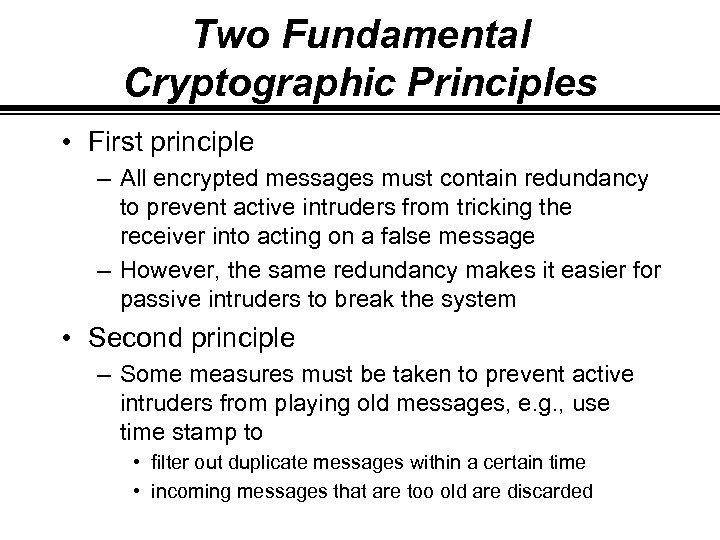Two Fundamental Cryptographic Principles • First principle – All encrypted messages must contain redundancy to prevent active intruders from tricking the receiver into acting on a false message – However, the same redundancy makes it easier for passive intruders to break the system • Second principle – Some measures must be taken to prevent active intruders from playing old messages, e. g. , use time stamp to • filter out duplicate messages within a certain time • incoming messages that are too old are discarded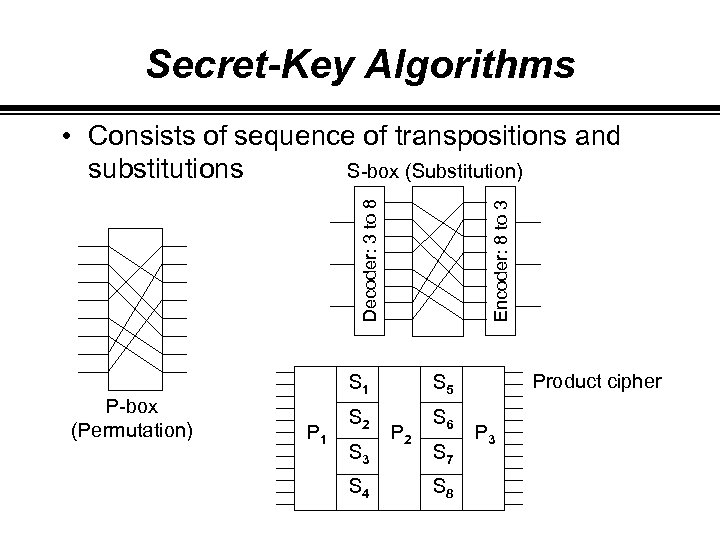Secret-Key Algorithms P-box (Permutation) Encoder: 8 to 3 Decoder: 3 to 8 • Consists of sequence of transpositions and S-box (Substitution) substitutions S 1 P 1 S 2 S 6 Product cipher S 5 S 3 S 4 P 2 S 7 S 8 P 3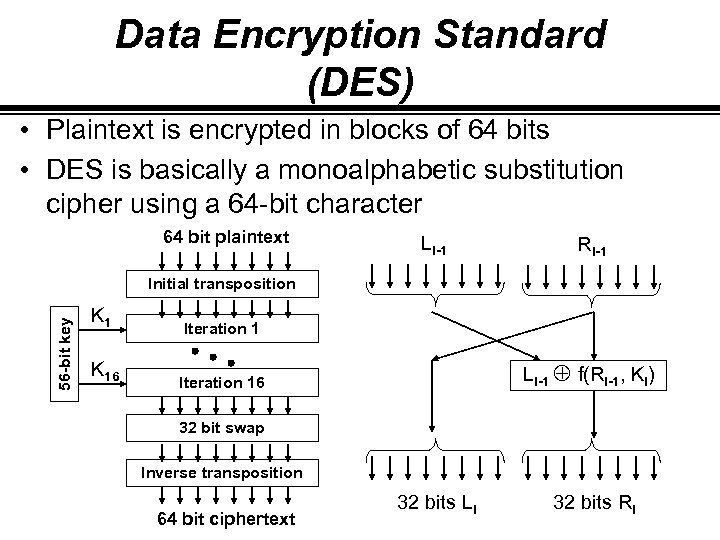Data Encryption Standard (DES) • Plaintext is encrypted in blocks of 64 bits • DES is basically a monoalphabetic substitution cipher using a 64 -bit character 64 bit plaintext Li-1 Ri-1 56 -bit key Initial transposition K 1 Iteration 1 K 16 Iteration 16 Li-1 Å f(Ri-1, Ki) 32 bit swap Inverse transposition 64 bit ciphertext 32 bits Li 32 bits Ri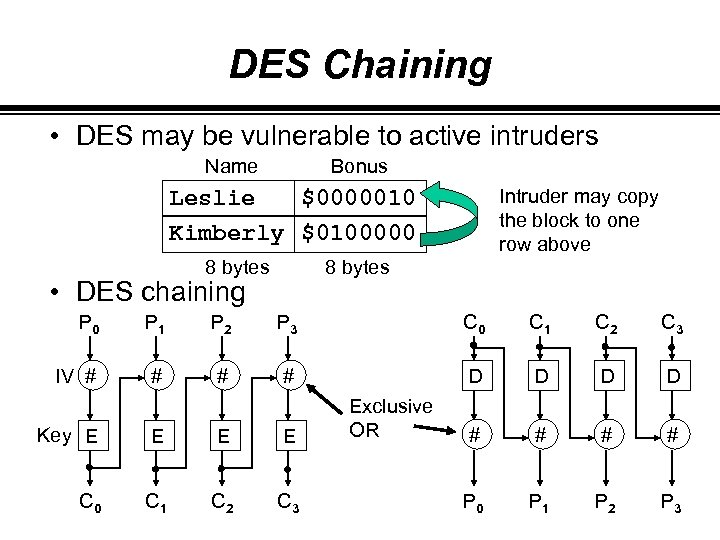DES Chaining • DES may be vulnerable to active intruders Name Bonus Leslie \$0000010 Kimberly \$0100000 8 bytes Intruder may copy the block to one row above 8 bytes • DES chaining P 0 P 1 P 2 P 3 C 0 C 1 C 2 C 3 IV # # D D # # P 0 P 1 P 2 P 3 Key E E C 0 C 1 C 2 C 3 Exclusive OR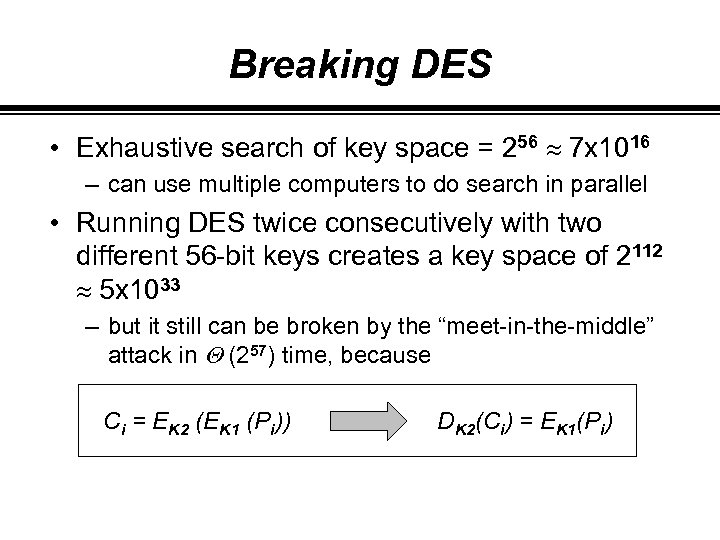Breaking DES • Exhaustive search of key space = 256 » 7 x 1016 – can use multiple computers to do search in parallel • Running DES twice consecutively with two different 56 -bit keys creates a key space of 2112 » 5 x 1033 – but it still can be broken by the “meet-in-the-middle” attack in Q (257) time, because Ci = EK 2 (EK 1 (Pi)) DK 2(Ci) = EK 1(Pi)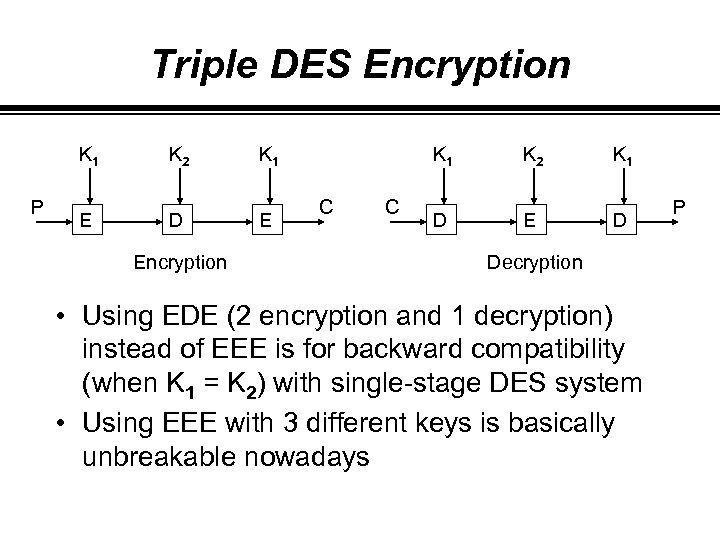Triple DES Encryption K 1 P K 2 K 1 E D E Encryption K 1 C C K 2 K 1 D E D Decryption • Using EDE (2 encryption and 1 decryption) instead of EEE is for backward compatibility (when K 1 = K 2) with single-stage DES system • Using EEE with 3 different keys is basically unbreakable nowadays P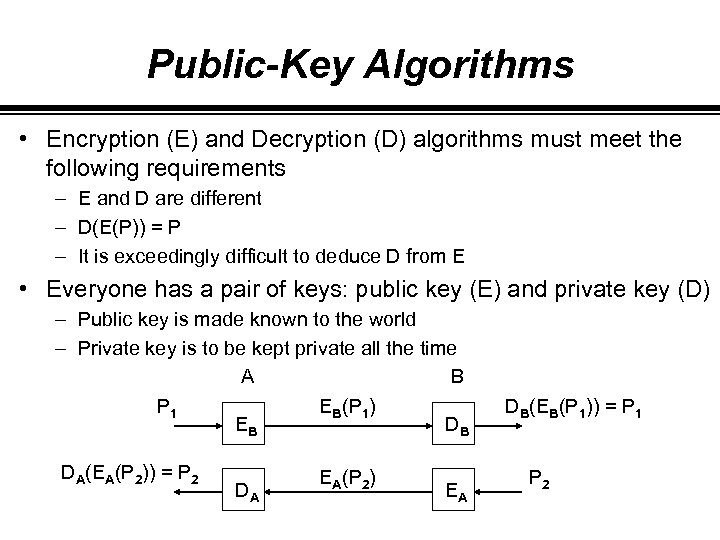Public-Key Algorithms • Encryption (E) and Decryption (D) algorithms must meet the following requirements – E and D are different – D(E(P)) = P – It is exceedingly difficult to deduce D from E • Everyone has a pair of keys: public key (E) and private key (D) – Public key is made known to the world – Private key is to be kept private all the time A B P 1 EB(P 1) EB DB DA(EA(P 2)) = P 2 DA EA(P 2) EA DB(EB(P 1)) = P 1 P 2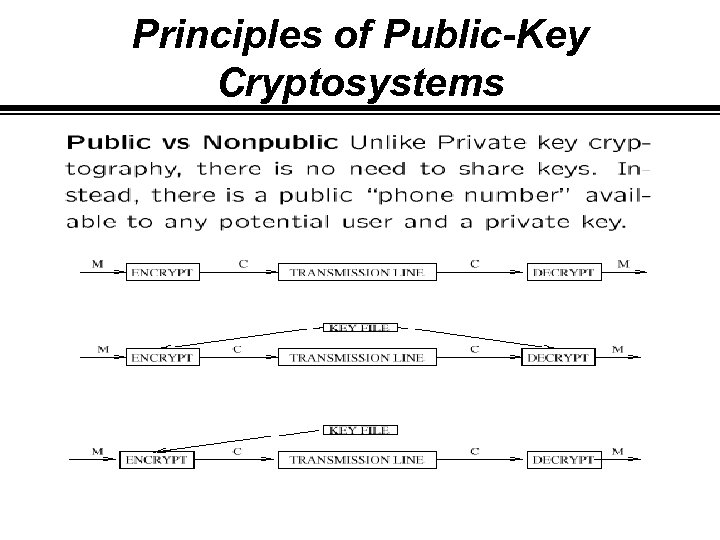Principles of Public-Key Cryptosystems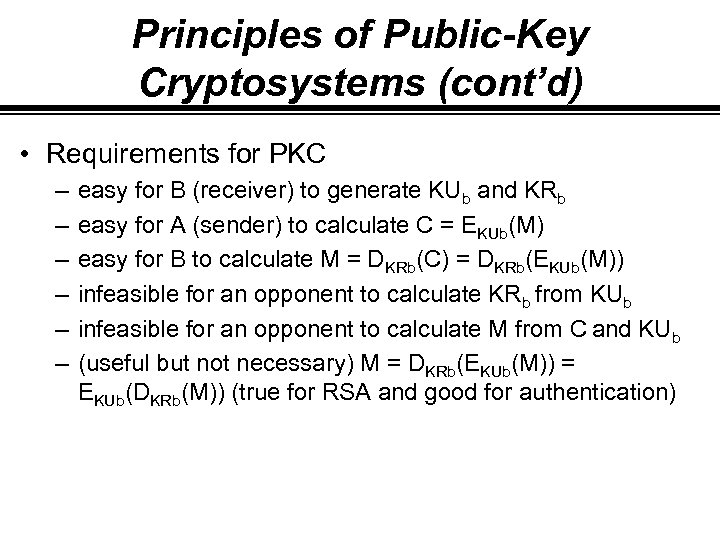Principles of Public-Key Cryptosystems (cont’d) • Requirements for PKC – – – easy for B (receiver) to generate KUb and KRb easy for A (sender) to calculate C = EKUb(M) easy for B to calculate M = DKRb(C) = DKRb(EKUb(M)) infeasible for an opponent to calculate KRb from KUb infeasible for an opponent to calculate M from C and KUb (useful but not necessary) M = DKRb(EKUb(M)) = EKUb(DKRb(M)) (true for RSA and good for authentication)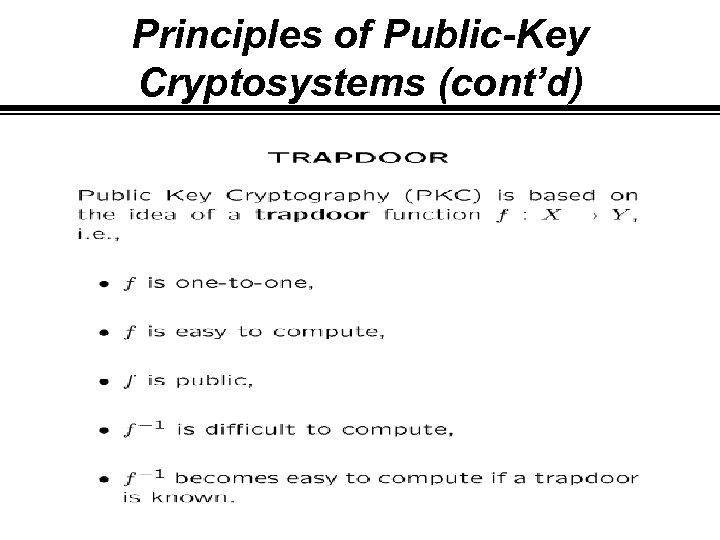Principles of Public-Key Cryptosystems (cont’d)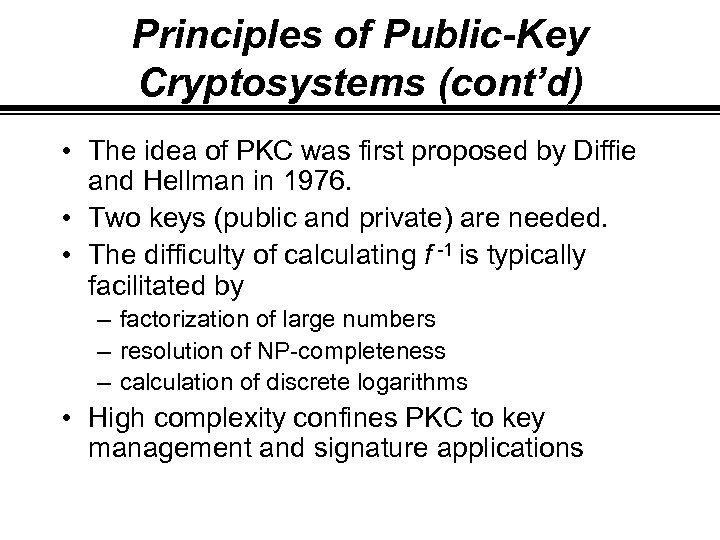Principles of Public-Key Cryptosystems (cont’d) • The idea of PKC was first proposed by Diffie and Hellman in 1976. • Two keys (public and private) are needed. • The difficulty of calculating f -1 is typically facilitated by – factorization of large numbers – resolution of NP-completeness – calculation of discrete logarithms • High complexity confines PKC to key management and signature applications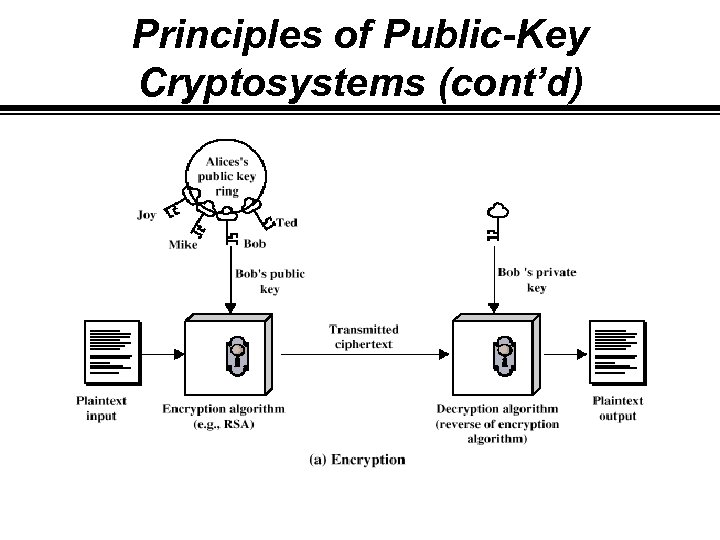Principles of Public-Key Cryptosystems (cont’d)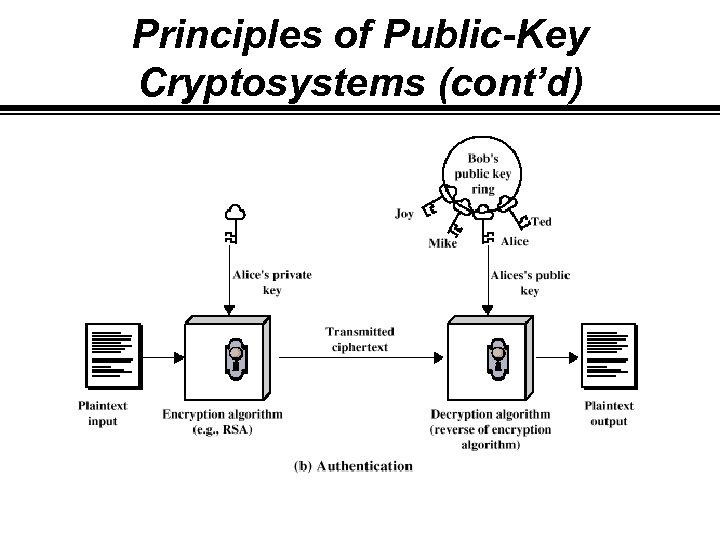Principles of Public-Key Cryptosystems (cont’d)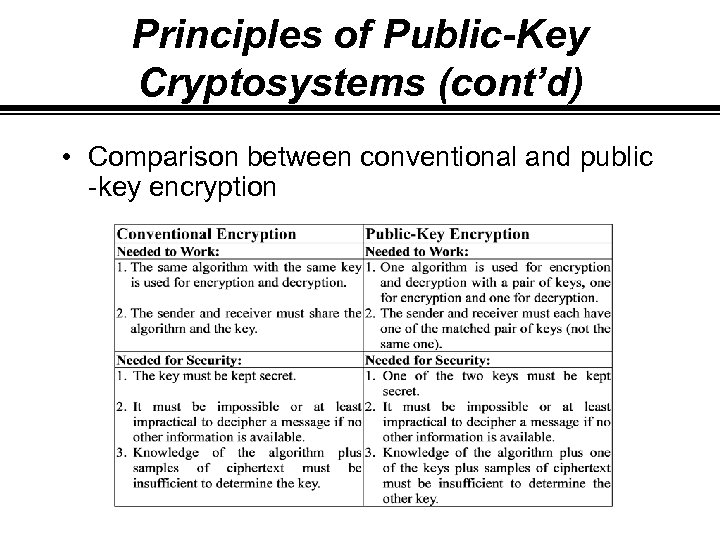Principles of Public-Key Cryptosystems (cont’d) • Comparison between conventional and public -key encryption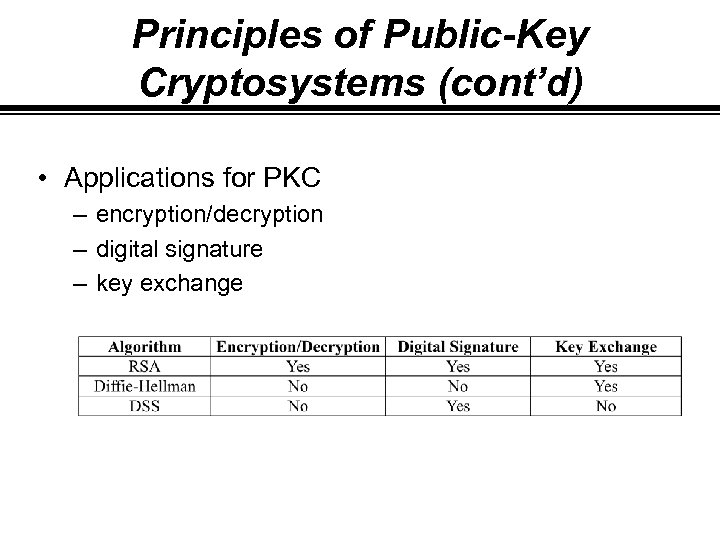Principles of Public-Key Cryptosystems (cont’d) • Applications for PKC – encryption/decryption – digital signature – key exchange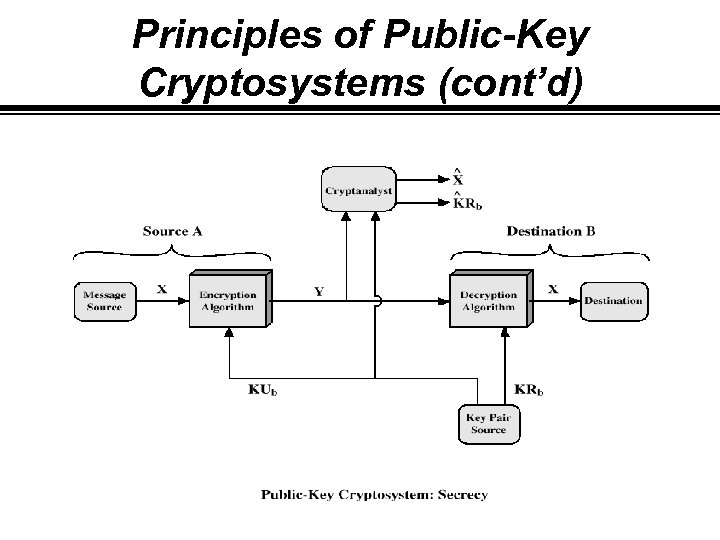Principles of Public-Key Cryptosystems (cont’d)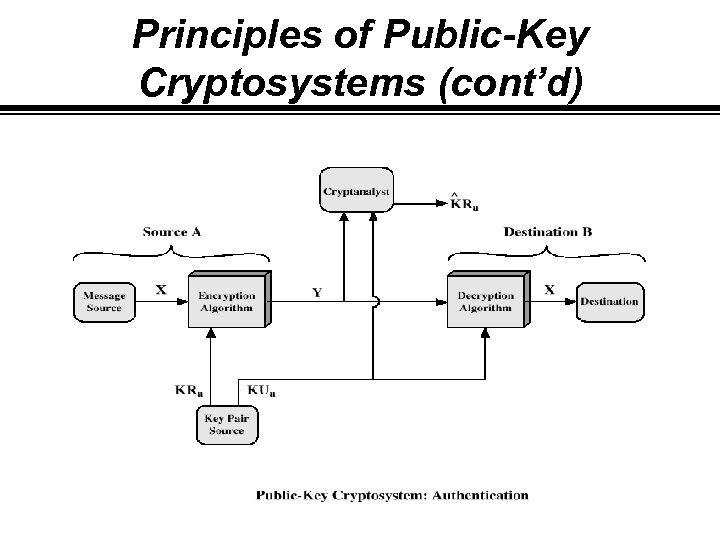Principles of Public-Key Cryptosystems (cont’d)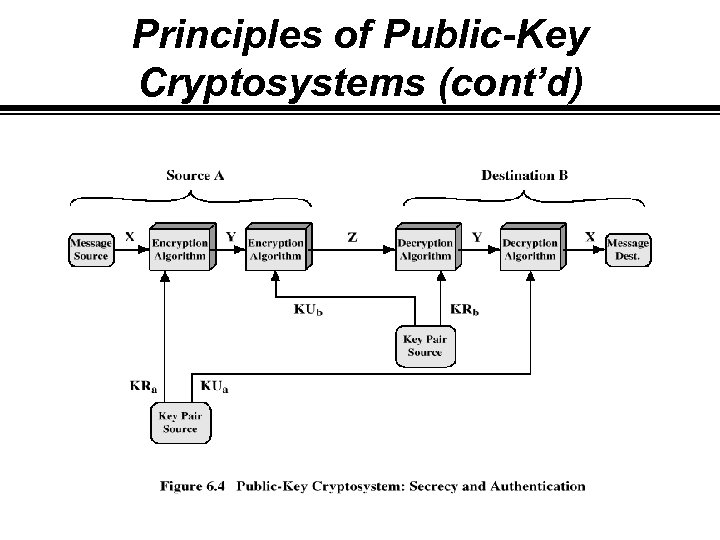Principles of Public-Key Cryptosystems (cont’d)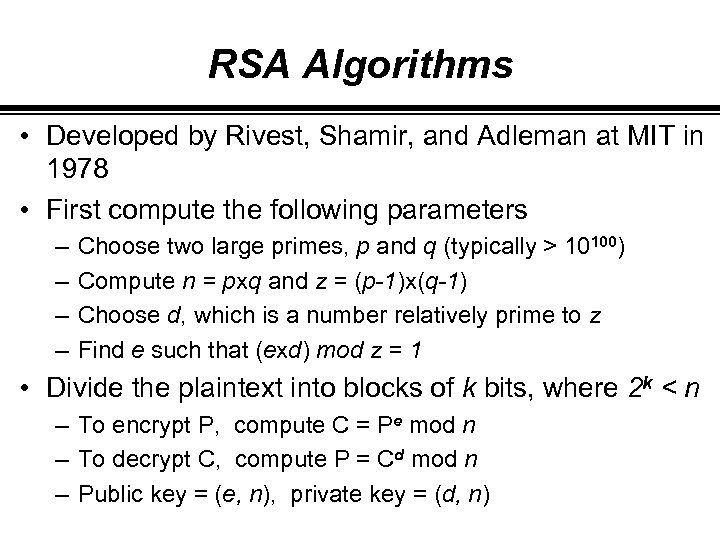RSA Algorithms • Developed by Rivest, Shamir, and Adleman at MIT in 1978 • First compute the following parameters – – Choose two large primes, p and q (typically > 10100) Compute n = pxq and z = (p-1)x(q-1) Choose d, which is a number relatively prime to z Find e such that (exd) mod z = 1 • Divide the plaintext into blocks of k bits, where 2 k < n – To encrypt P, compute C = Pe mod n – To decrypt C, compute P = Cd mod n – Public key = (e, n), private key = (d, n)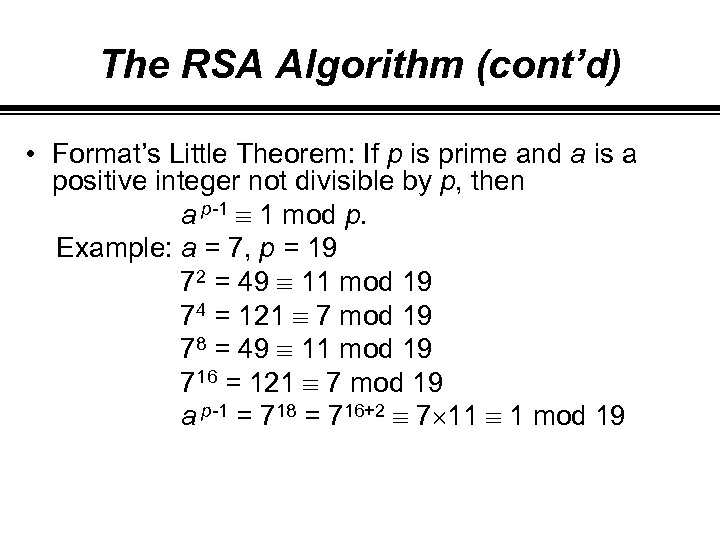The RSA Algorithm (cont’d) • Format’s Little Theorem: If p is prime and a is a positive integer not divisible by p, then a p-1 1 mod p. Example: a = 7, p = 19 72 = 49 11 mod 19 74 = 121 7 mod 19 78 = 49 11 mod 19 716 = 121 7 mod 19 a p-1 = 718 = 716+2 7 11 1 mod 19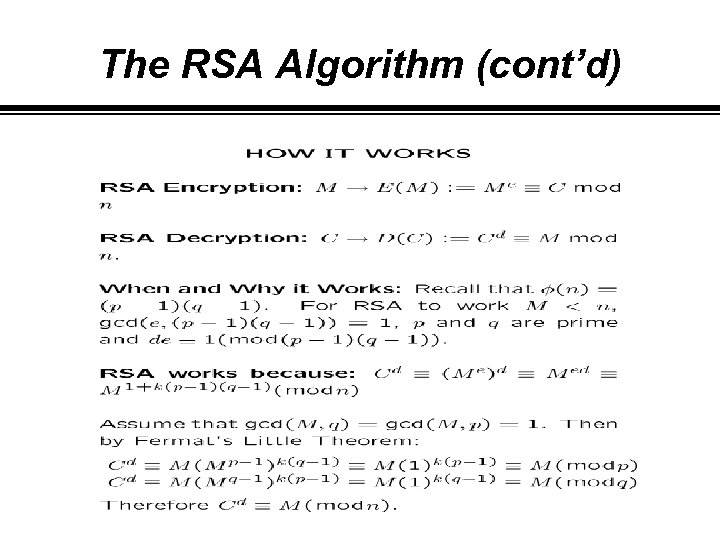The RSA Algorithm (cont’d)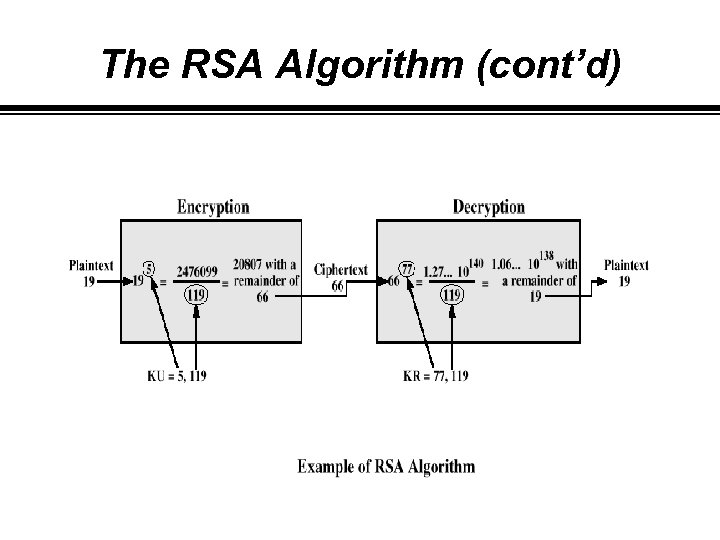The RSA Algorithm (cont’d)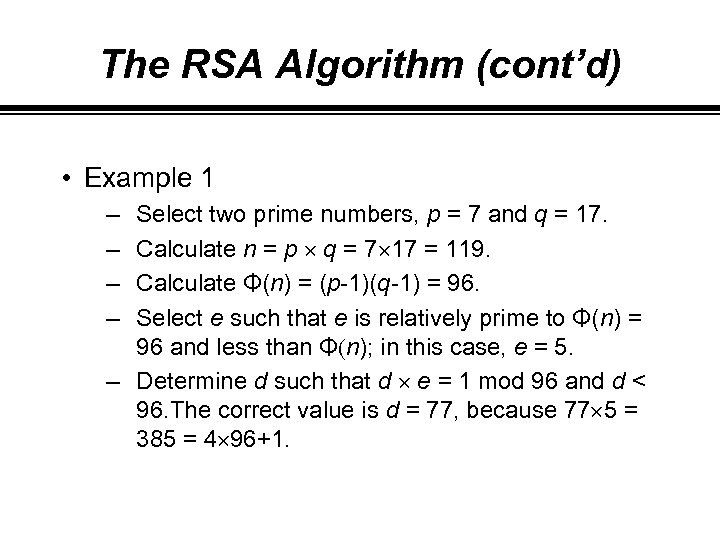The RSA Algorithm (cont’d) • Example 1 – – Select two prime numbers, p = 7 and q = 17. Calculate n = p q = 7 17 = 119. Calculate Φ(n) = (p-1)(q-1) = 96. Select e such that e is relatively prime to Φ(n) = 96 and less than Φ(n); in this case, e = 5. – Determine d such that d e = 1 mod 96 and d < 96. The correct value is d = 77, because 77 5 = 385 = 4 96+1.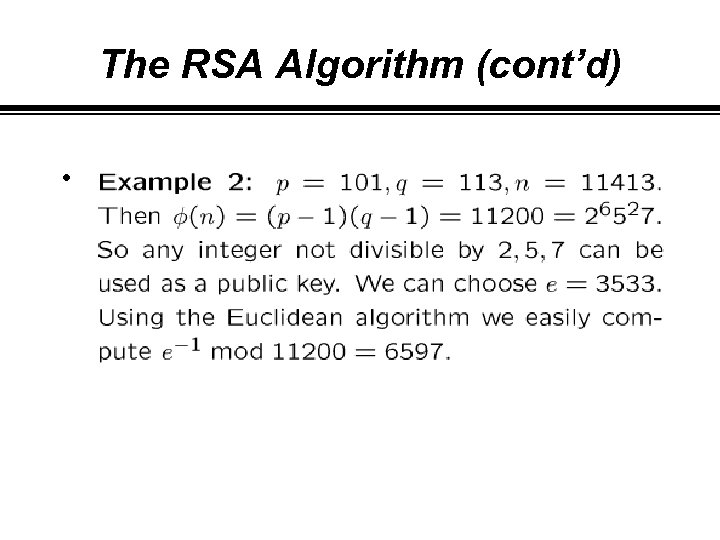The RSA Algorithm (cont’d) •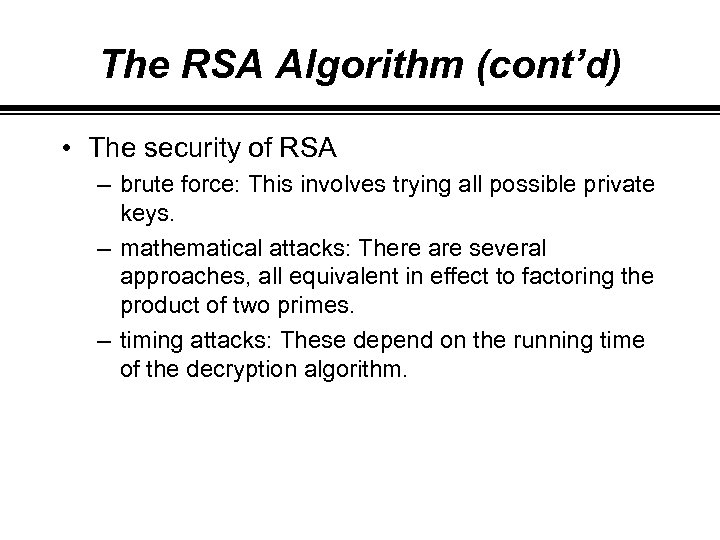The RSA Algorithm (cont’d) • The security of RSA – brute force: This involves trying all possible private keys. – mathematical attacks: There are several approaches, all equivalent in effect to factoring the product of two primes. – timing attacks: These depend on the running time of the decryption algorithm.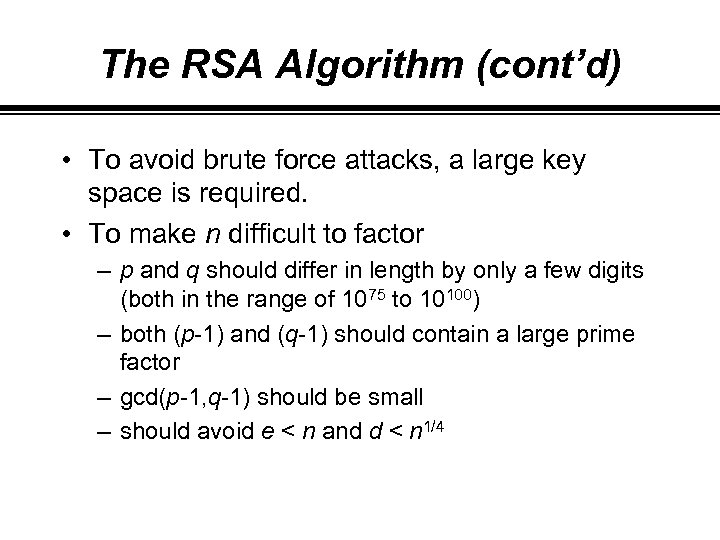The RSA Algorithm (cont’d) • To avoid brute force attacks, a large key space is required. • To make n difficult to factor – p and q should differ in length by only a few digits (both in the range of 1075 to 10100) – both (p-1) and (q-1) should contain a large prime factor – gcd(p-1, q-1) should be small – should avoid e < n and d < n 1/4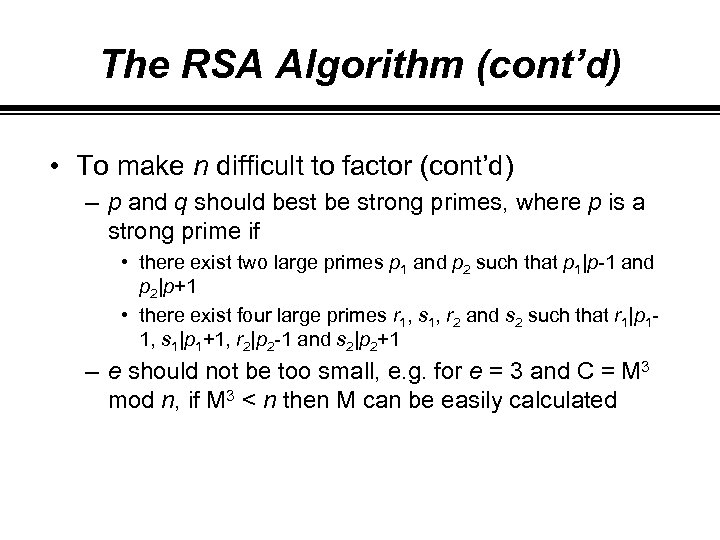The RSA Algorithm (cont’d) • To make n difficult to factor (cont’d) – p and q should best be strong primes, where p is a strong prime if • there exist two large primes p 1 and p 2 such that p 1|p-1 and p 2|p+1 • there exist four large primes r 1, s 1, r 2 and s 2 such that r 1|p 11, s 1|p 1+1, r 2|p 2 -1 and s 2|p 2+1 – e should not be too small, e. g. for e = 3 and C = M 3 mod n, if M 3 < n then M can be easily calculated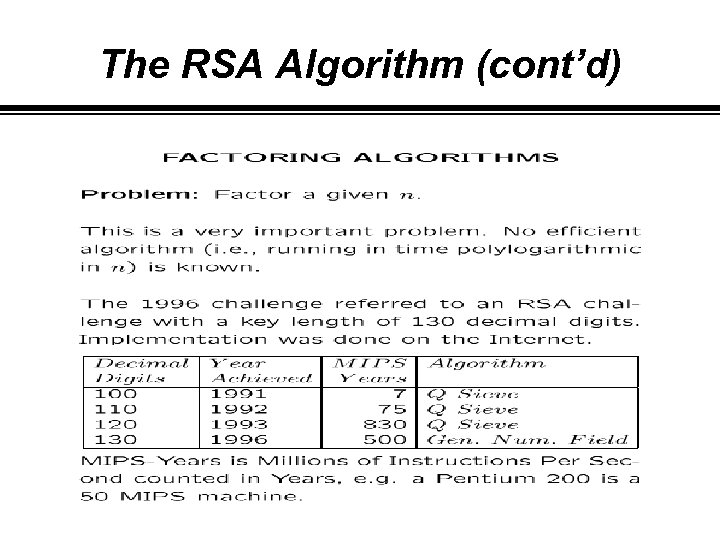The RSA Algorithm (cont’d)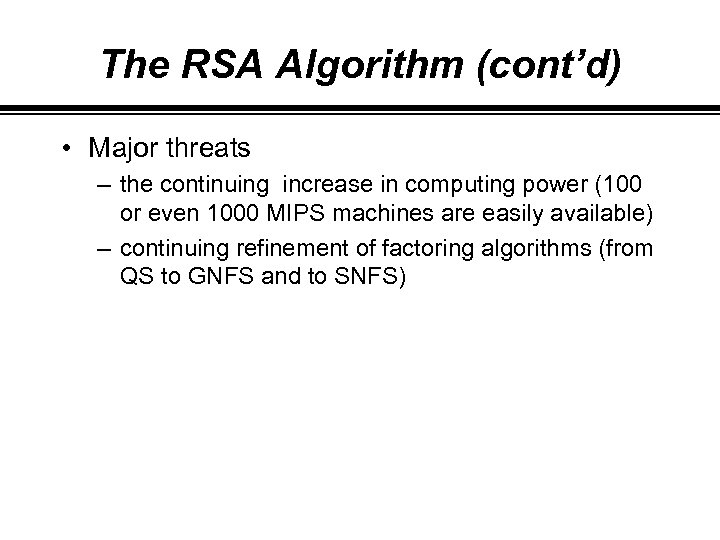The RSA Algorithm (cont’d) • Major threats – the continuing increase in computing power (100 or even 1000 MIPS machines are easily available) – continuing refinement of factoring algorithms (from QS to GNFS and to SNFS)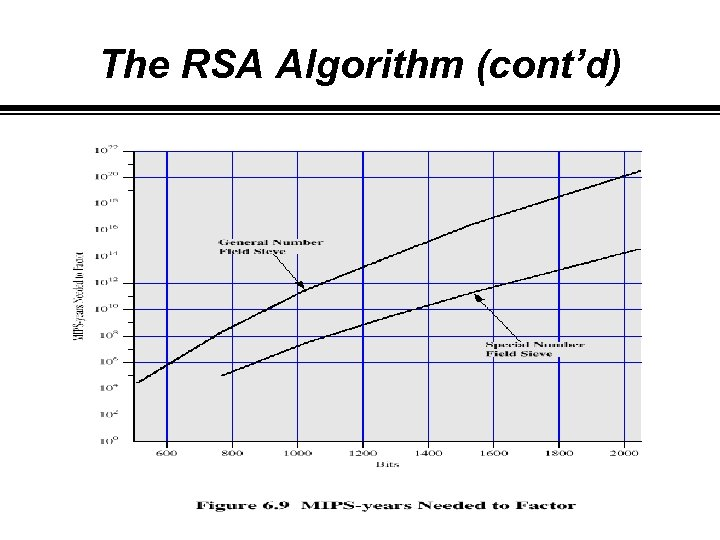The RSA Algorithm (cont’d)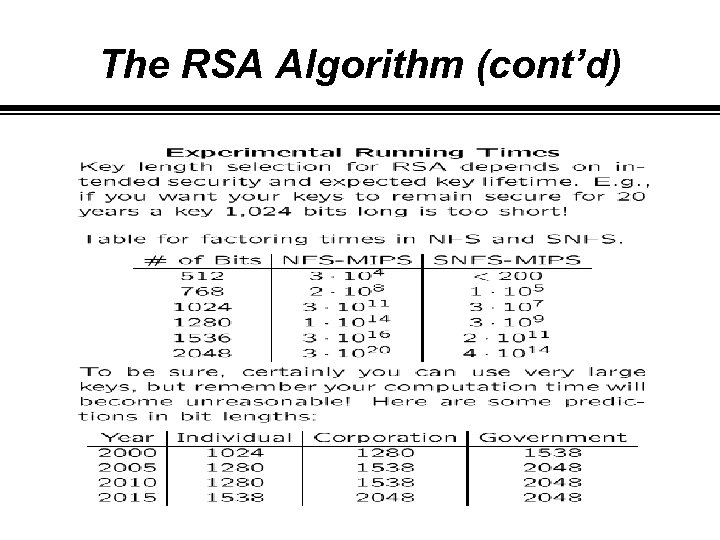The RSA Algorithm (cont’d)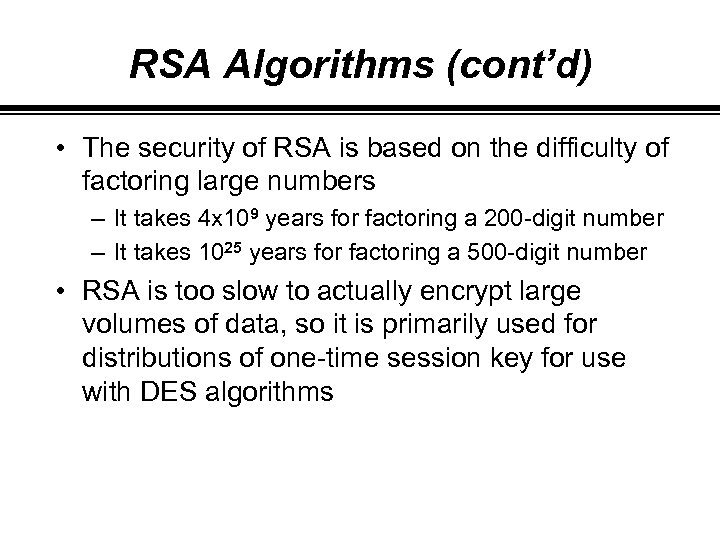RSA Algorithms (cont’d) • The security of RSA is based on the difficulty of factoring large numbers – It takes 4 x 109 years for factoring a 200 -digit number – It takes 1025 years for factoring a 500 -digit number • RSA is too slow to actually encrypt large volumes of data, so it is primarily used for distributions of one-time session key for use with DES algorithms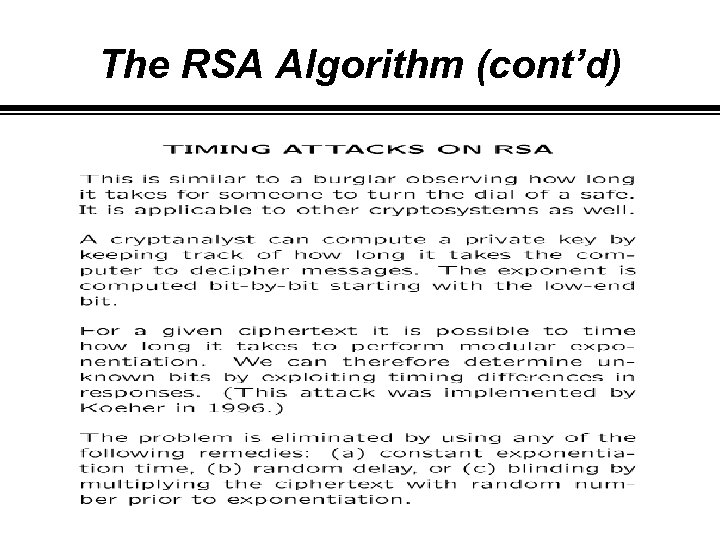The RSA Algorithm (cont’d)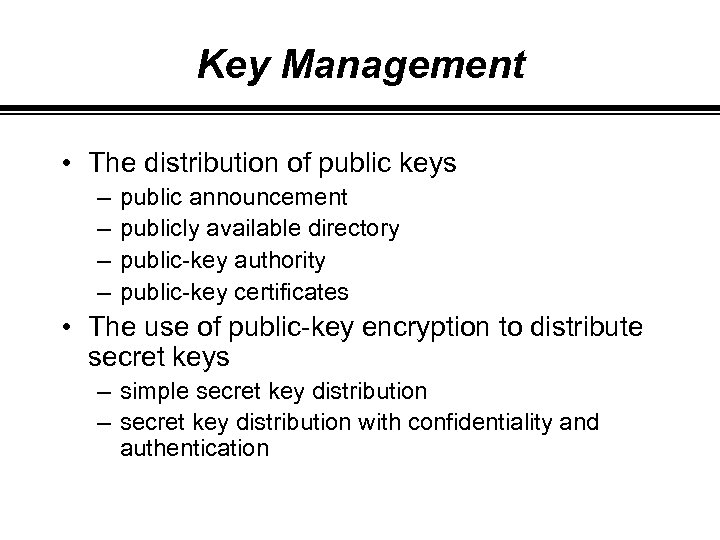Key Management • The distribution of public keys – – public announcement publicly available directory public-key authority public-key certificates • The use of public-key encryption to distribute secret keys – simple secret key distribution – secret key distribution with confidentiality and authentication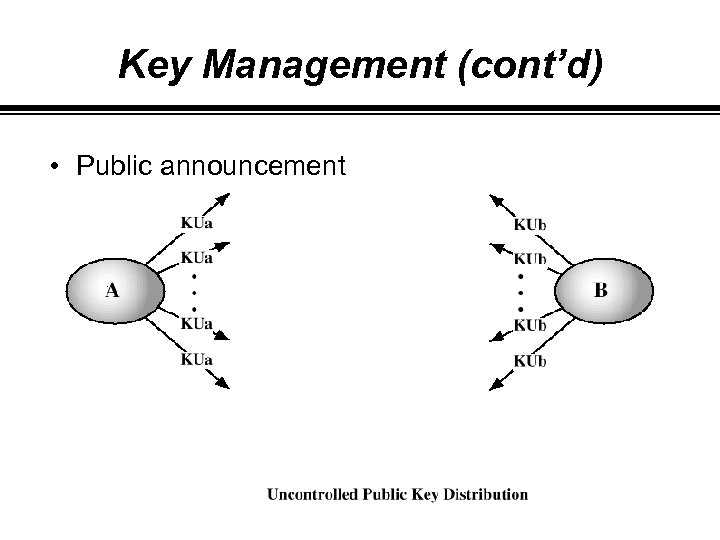Key Management (cont’d) • Public announcement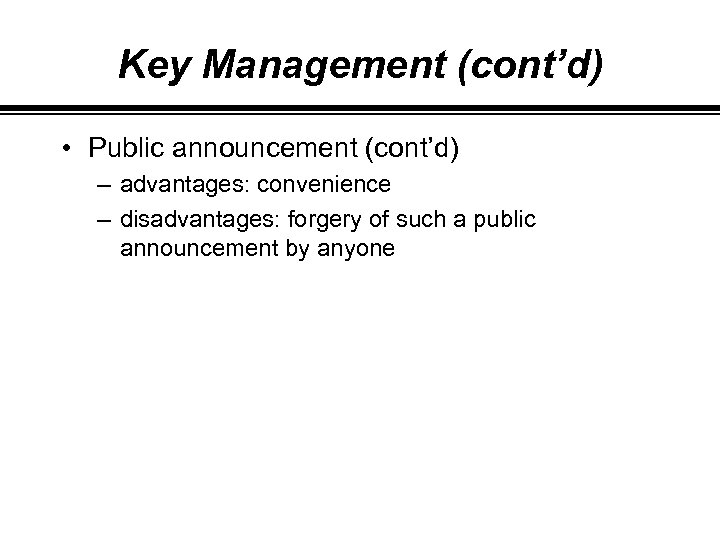Key Management (cont’d) • Public announcement (cont’d) – advantages: convenience – disadvantages: forgery of such a public announcement by anyone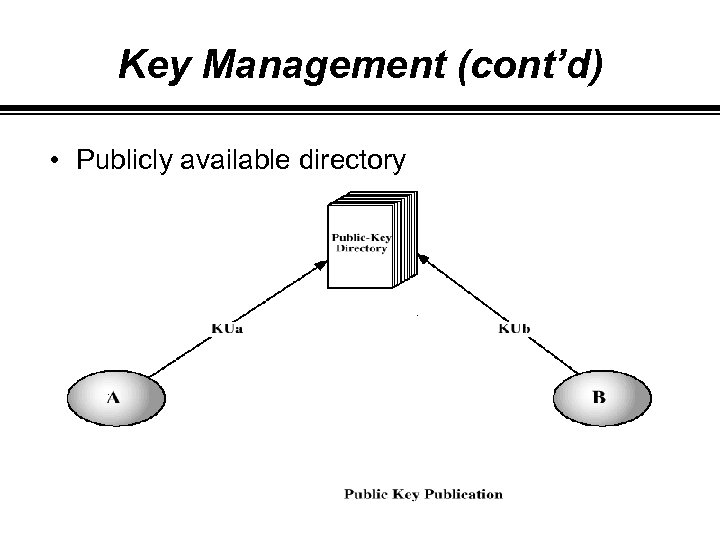Key Management (cont’d) • Publicly available directory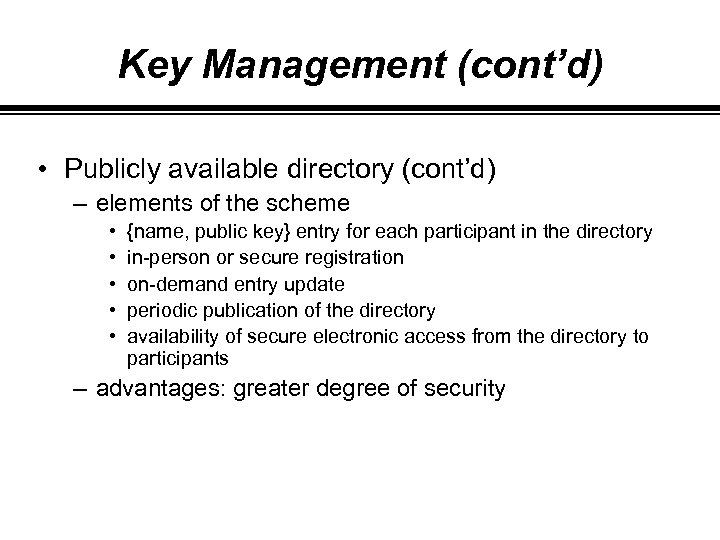Key Management (cont’d) • Publicly available directory (cont’d) – elements of the scheme • • • {name, public key} entry for each participant in the directory in-person or secure registration on-demand entry update periodic publication of the directory availability of secure electronic access from the directory to participants – advantages: greater degree of security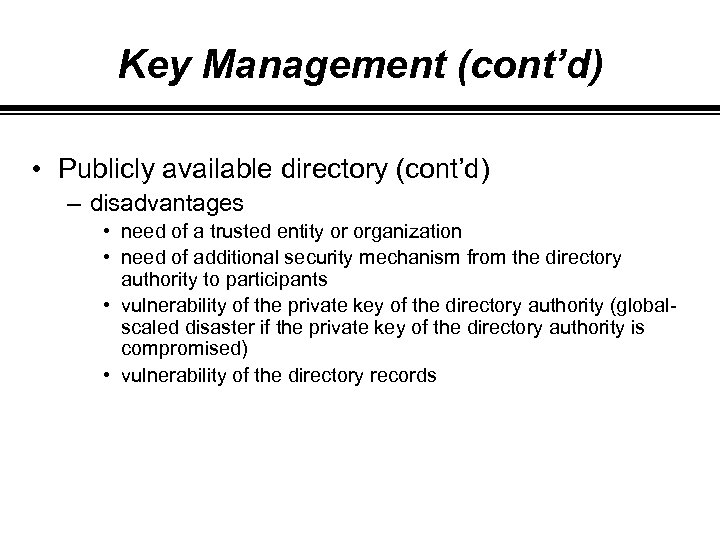Key Management (cont’d) • Publicly available directory (cont’d) – disadvantages • need of a trusted entity or organization • need of additional security mechanism from the directory authority to participants • vulnerability of the private key of the directory authority (globalscaled disaster if the private key of the directory authority is compromised) • vulnerability of the directory records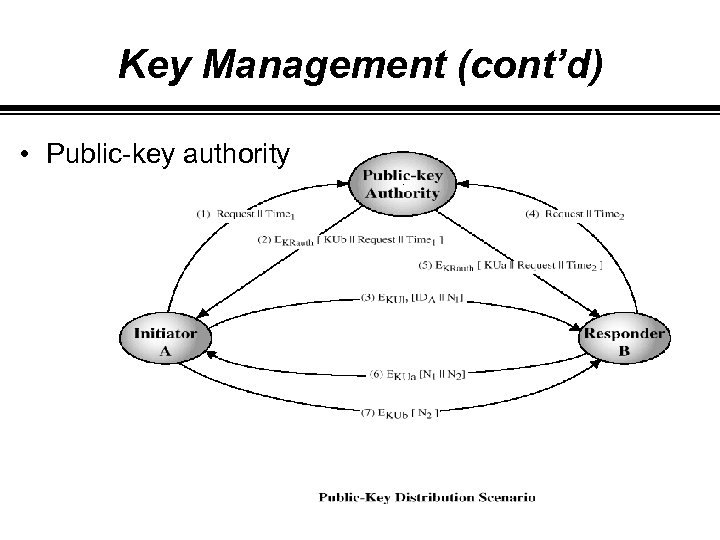Key Management (cont’d) • Public-key authority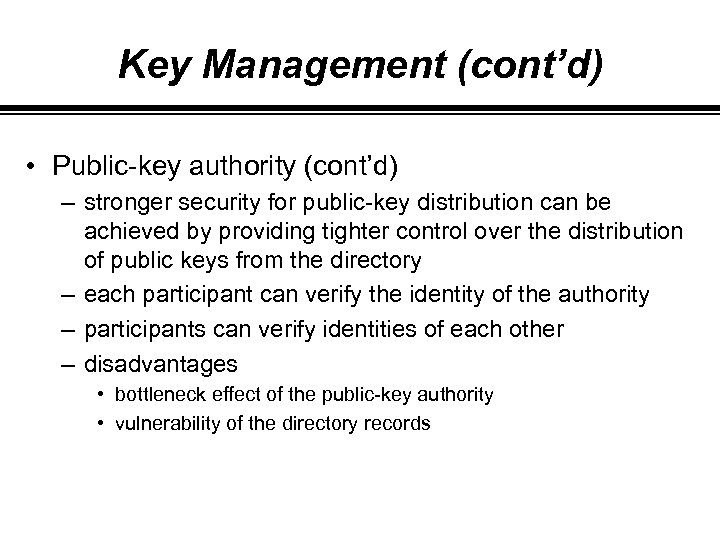Key Management (cont’d) • Public-key authority (cont’d) – stronger security for public-key distribution can be achieved by providing tighter control over the distribution of public keys from the directory – each participant can verify the identity of the authority – participants can verify identities of each other – disadvantages • bottleneck effect of the public-key authority • vulnerability of the directory records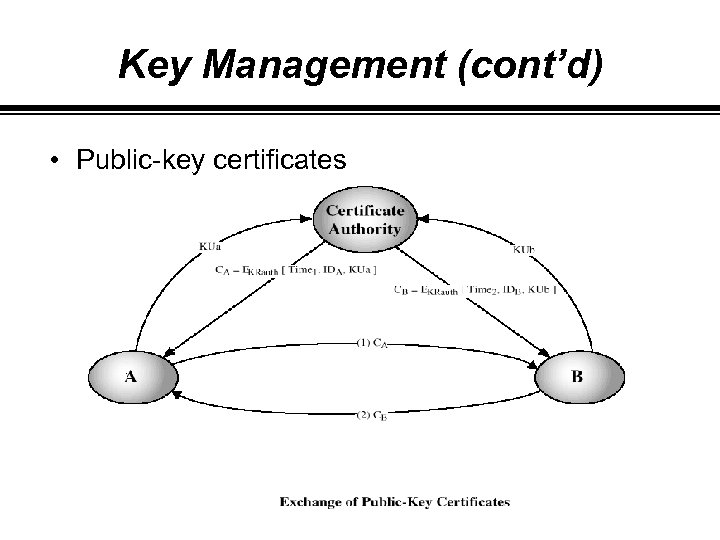Key Management (cont’d) • Public-key certificates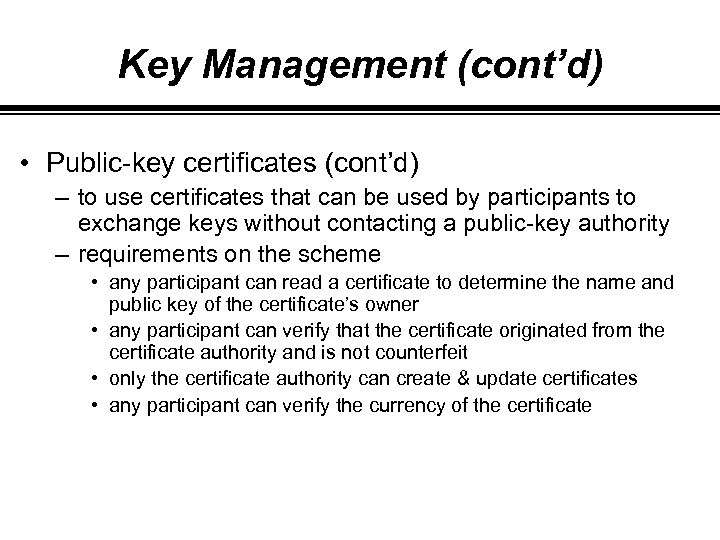Key Management (cont’d) • Public-key certificates (cont’d) – to use certificates that can be used by participants to exchange keys without contacting a public-key authority – requirements on the scheme • any participant can read a certificate to determine the name and public key of the certificate’s owner • any participant can verify that the certificate originated from the certificate authority and is not counterfeit • only the certificate authority can create & update certificates • any participant can verify the currency of the certificate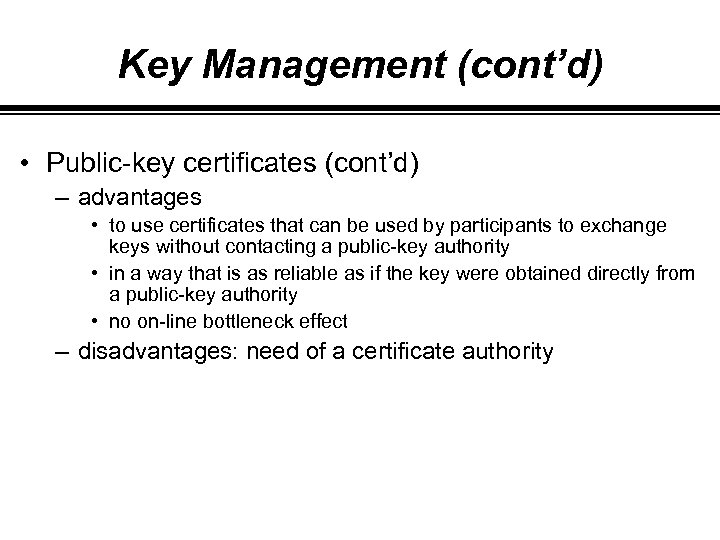Key Management (cont’d) • Public-key certificates (cont’d) – advantages • to use certificates that can be used by participants to exchange keys without contacting a public-key authority • in a way that is as reliable as if the key were obtained directly from a public-key authority • no on-line bottleneck effect – disadvantages: need of a certificate authority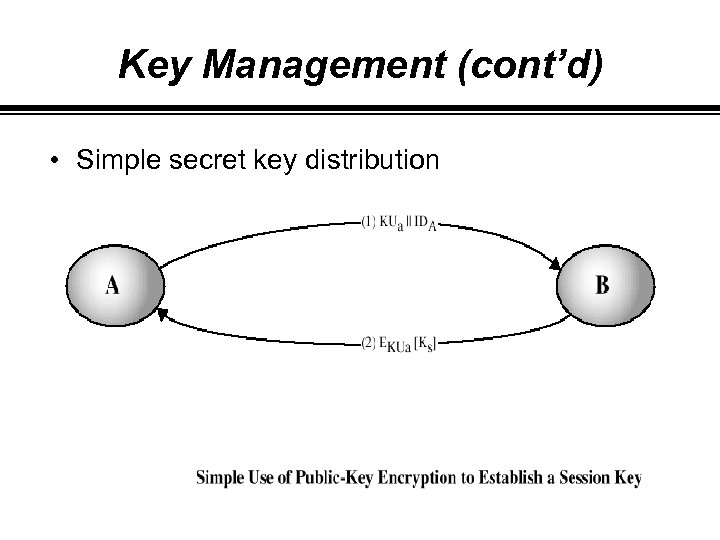Key Management (cont’d) • Simple secret key distribution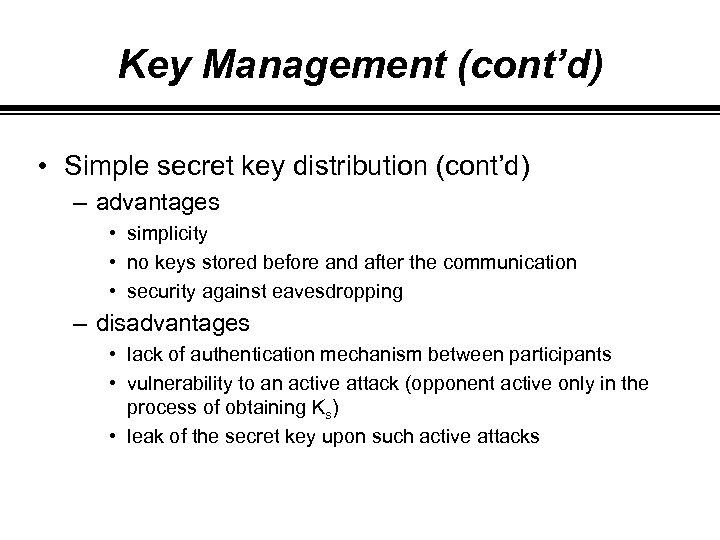Key Management (cont’d) • Simple secret key distribution (cont’d) – advantages • simplicity • no keys stored before and after the communication • security against eavesdropping – disadvantages • lack of authentication mechanism between participants • vulnerability to an active attack (opponent active only in the process of obtaining Ks) • leak of the secret key upon such active attacks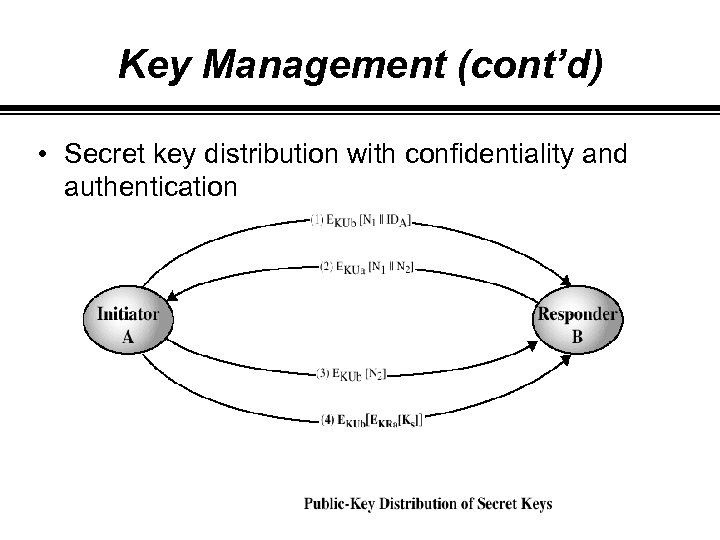Key Management (cont’d) • Secret key distribution with confidentiality and authentication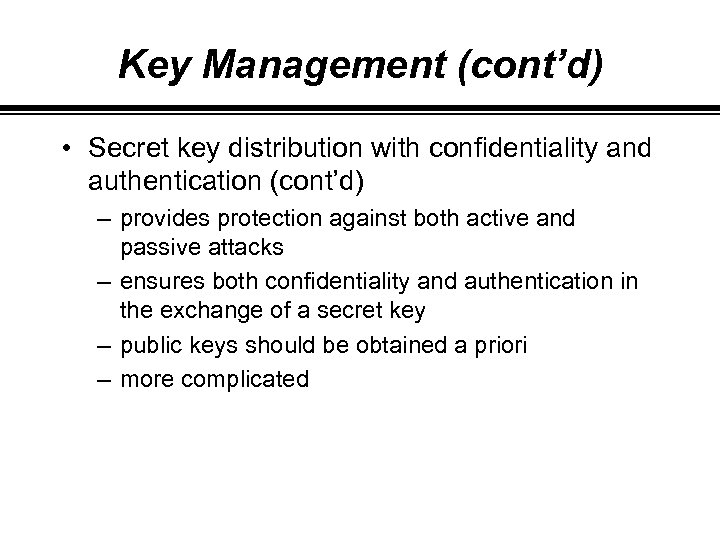Key Management (cont’d) • Secret key distribution with confidentiality and authentication (cont’d) – provides protection against both active and passive attacks – ensures both confidentiality and authentication in the exchange of a secret key – public keys should be obtained a priori – more complicated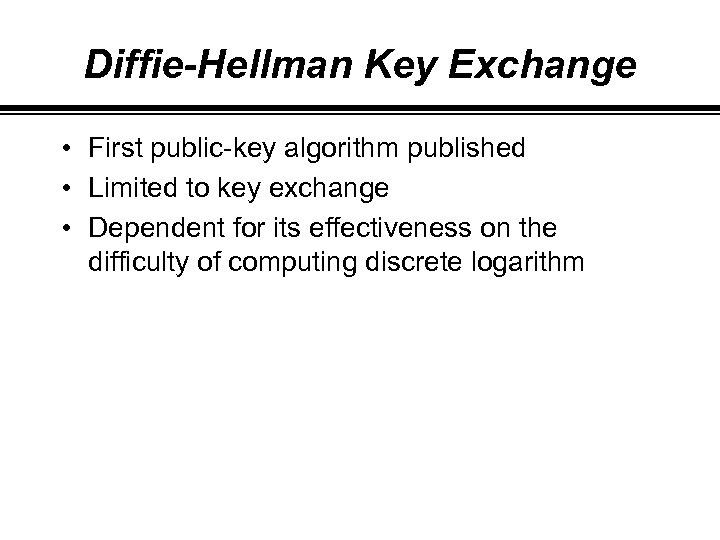Diffie-Hellman Key Exchange • First public-key algorithm published • Limited to key exchange • Dependent for its effectiveness on the difficulty of computing discrete logarithm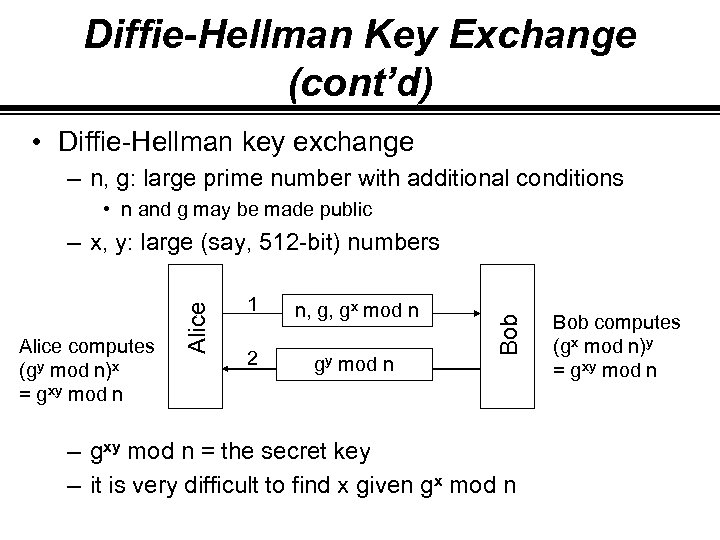Diffie-Hellman Key Exchange (cont’d) • Diffie-Hellman key exchange – n, g: large prime number with additional conditions • n and g may be made public 1 n, g, gx mod n 2 gy mod n Bob Alice computes (gy mod n)x = gxy mod n Alice – x, y: large (say, 512 -bit) numbers – gxy mod n = the secret key – it is very difficult to find x given gx mod n Bob computes (gx mod n)y = gxy mod n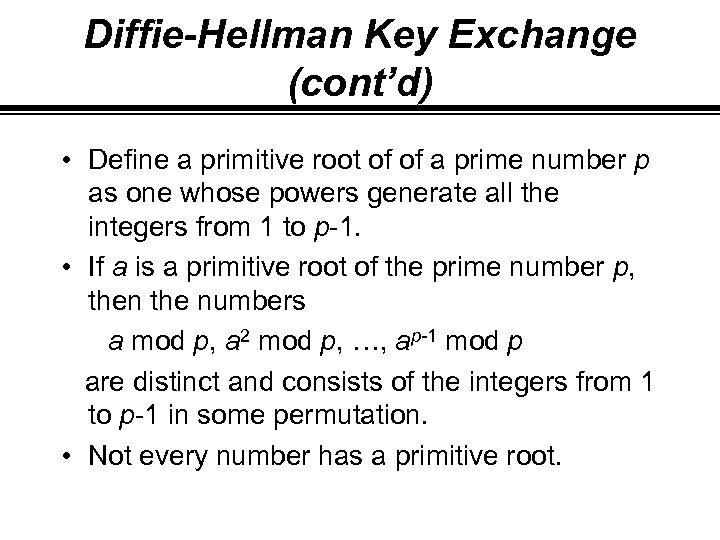Diffie-Hellman Key Exchange (cont’d) • Define a primitive root of of a prime number p as one whose powers generate all the integers from 1 to p-1. • If a is a primitive root of the prime number p, then the numbers a mod p, a 2 mod p, …, ap-1 mod p are distinct and consists of the integers from 1 to p-1 in some permutation. • Not every number has a primitive root.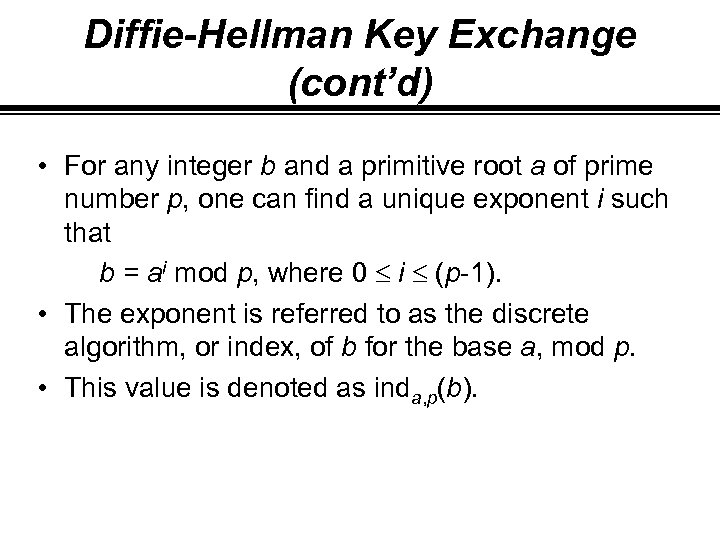Diffie-Hellman Key Exchange (cont’d) • For any integer b and a primitive root a of prime number p, one can find a unique exponent i such that b = ai mod p, where 0 i (p-1). • The exponent is referred to as the discrete algorithm, or index, of b for the base a, mod p. • This value is denoted as inda, p(b).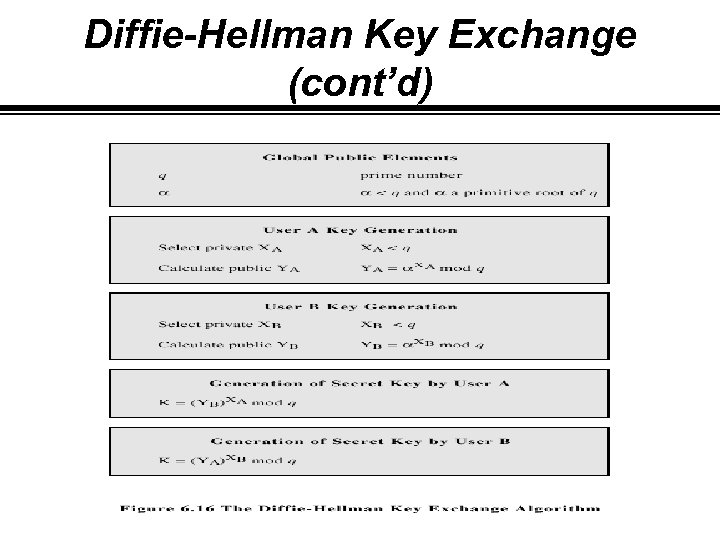Diffie-Hellman Key Exchange (cont’d)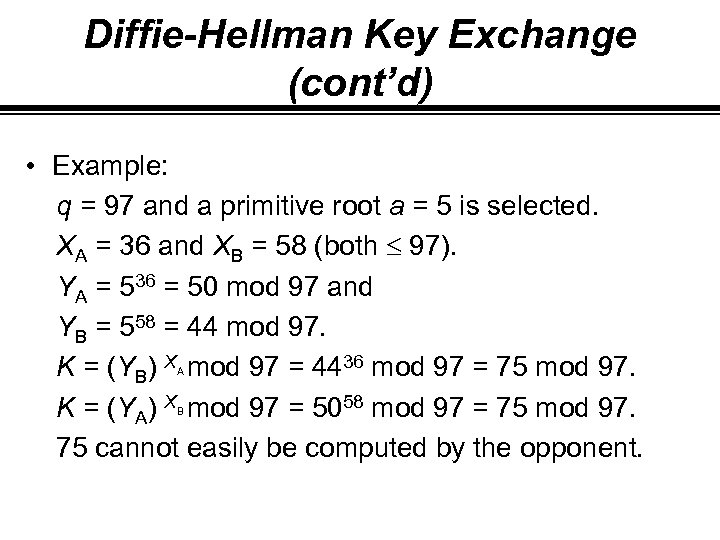Diffie-Hellman Key Exchange (cont’d) • Example: q = 97 and a primitive root a = 5 is selected. XA = 36 and XB = 58 (both 97). YA = 536 = 50 mod 97 and YB = 558 = 44 mod 97. K = (YB) X mod 97 = 4436 mod 97 = 75 mod 97. K = (YA) X mod 97 = 5058 mod 97 = 75 mod 97. 75 cannot easily be computed by the opponent. A B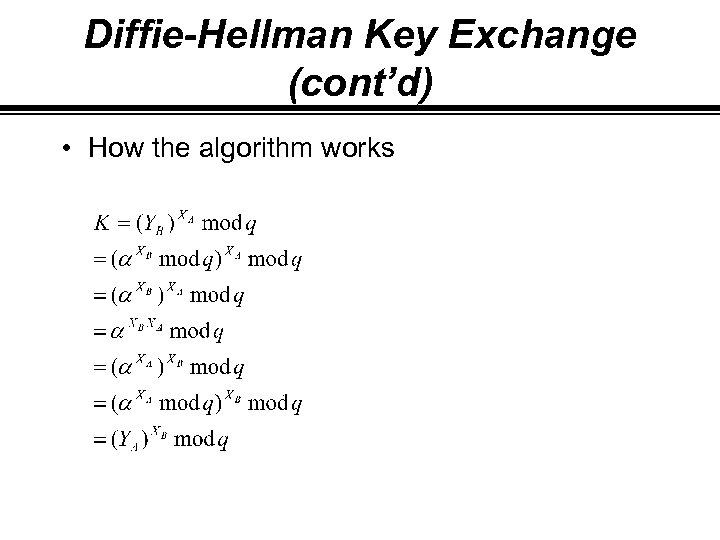Diffie-Hellman Key Exchange (cont’d) • How the algorithm works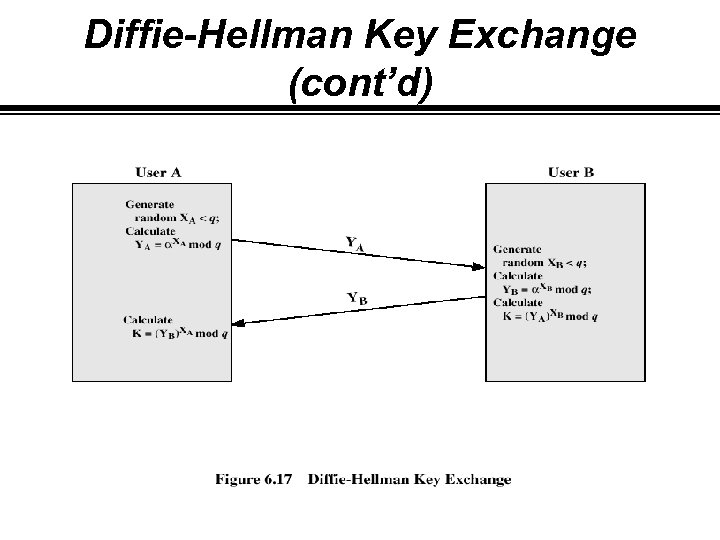Diffie-Hellman Key Exchange (cont’d)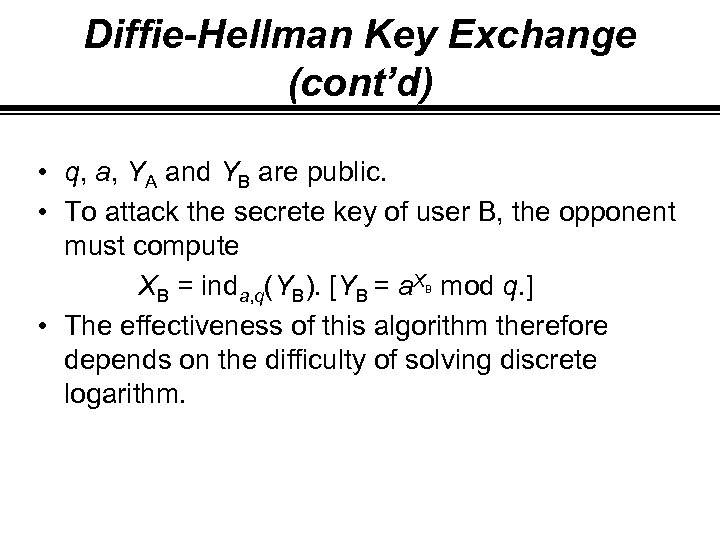Diffie-Hellman Key Exchange (cont’d) • q, a, YA and YB are public. • To attack the secrete key of user B, the opponent must compute XB = inda, q(YB). [YB = a. X mod q. ] • The effectiveness of this algorithm therefore depends on the difficulty of solving discrete logarithm. B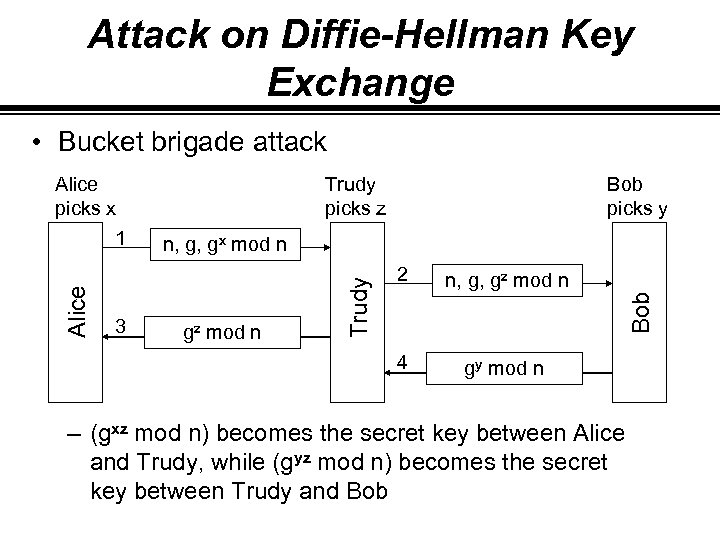Attack on Diffie-Hellman Key Exchange • Bucket brigade attack Alice picks x 1 Trudy picks z Bob picks y n, g, gx mod n gy mod n Bob Trudy Alice gz mod n n, g, gz mod n 4 3 2 – (gxz mod n) becomes the secret key between Alice and Trudy, while (gyz mod n) becomes the secret key between Trudy and Bob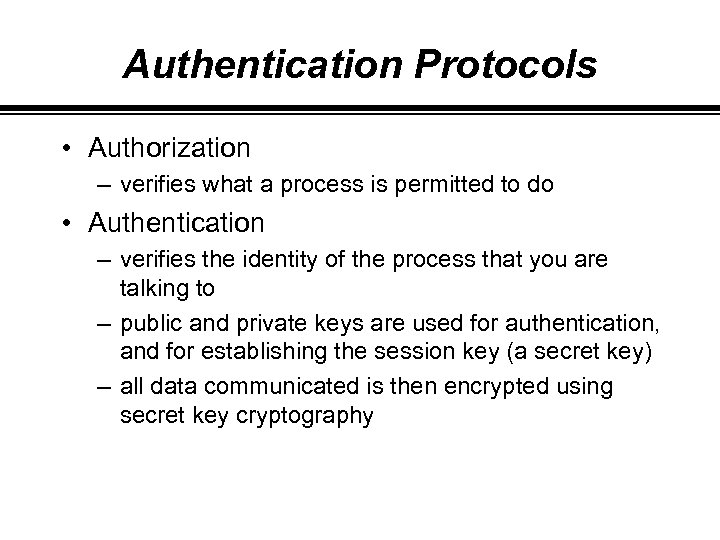Authentication Protocols • Authorization – verifies what a process is permitted to do • Authentication – verifies the identity of the process that you are talking to – public and private keys are used for authentication, and for establishing the session key (a secret key) – all data communicated is then encrypted using secret key cryptography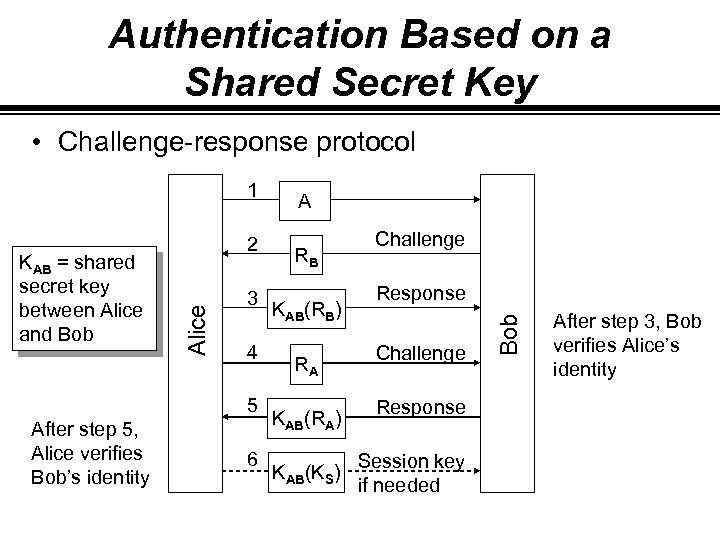Authentication Based on a Shared Secret Key • Challenge-response protocol Alice KAB = shared secret key between Alice and Bob 2 3 4 5 After step 5, Alice verifies Bob’s identity 6 A RB KAB(RB) RA KAB(RA) KAB(KS) Challenge Response Session key if needed Bob 1 After step 3, Bob verifies Alice’s identity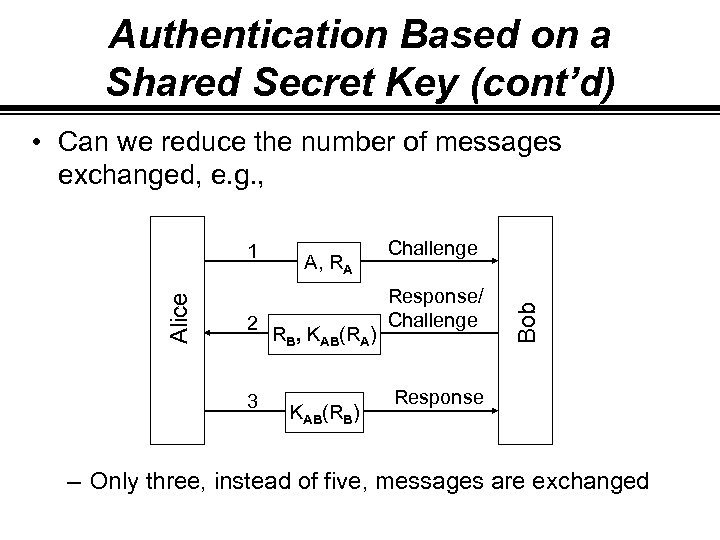Authentication Based on a Shared Secret Key (cont’d) • Can we reduce the number of messages exchanged, e. g. , 2 3 A, RA RB, KAB(RA) KAB(RB) Challenge Response/ Challenge Bob Alice 1 Response – Only three, instead of five, messages are exchanged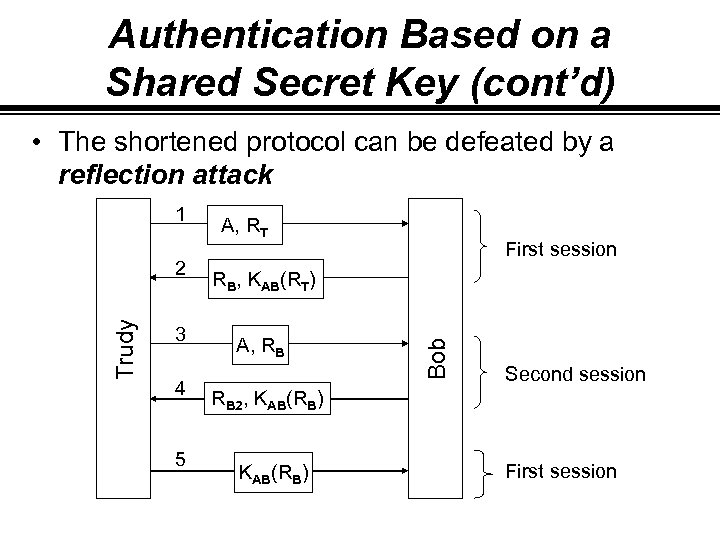Authentication Based on a Shared Secret Key (cont’d) • The shortened protocol can be defeated by a reflection attack Trudy 2 3 4 5 A, RT First session RB, KAB(RT) A, RB Bob 1 Second session RB 2, KAB(RB) First session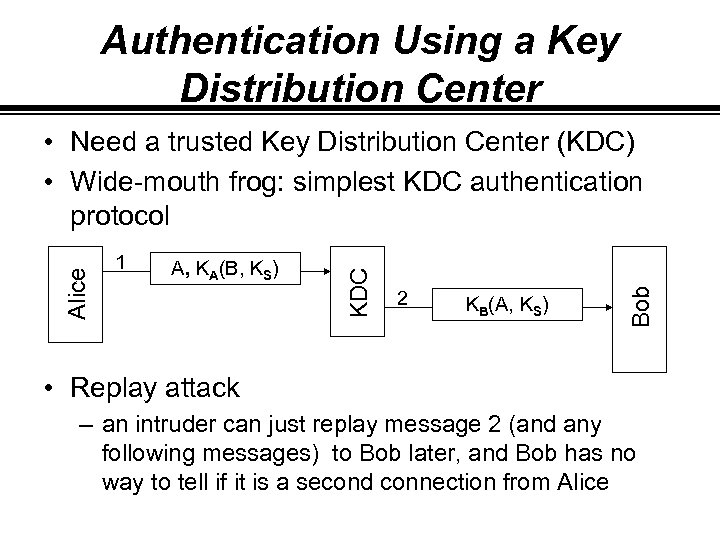Authentication Using a Key Distribution Center A, KA(B, KS) 2 KB(A, KS) Bob 1 KDC Alice • Need a trusted Key Distribution Center (KDC) • Wide-mouth frog: simplest KDC authentication protocol • Replay attack – an intruder can just replay message 2 (and any following messages) to Bob later, and Bob has no way to tell if it is a second connection from Alice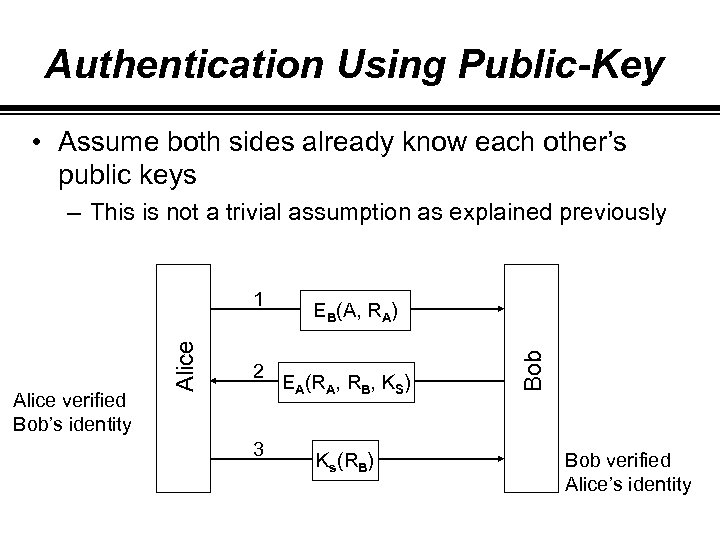Authentication Using Public-Key • Assume both sides already know each other’s public keys – This is not a trivial assumption as explained previously 2 3 EB(A, RA) EA(RA, RB, KS) Ks(RB) Bob Alice verified Bob’s identity Alice 1 Bob verified Alice’s identity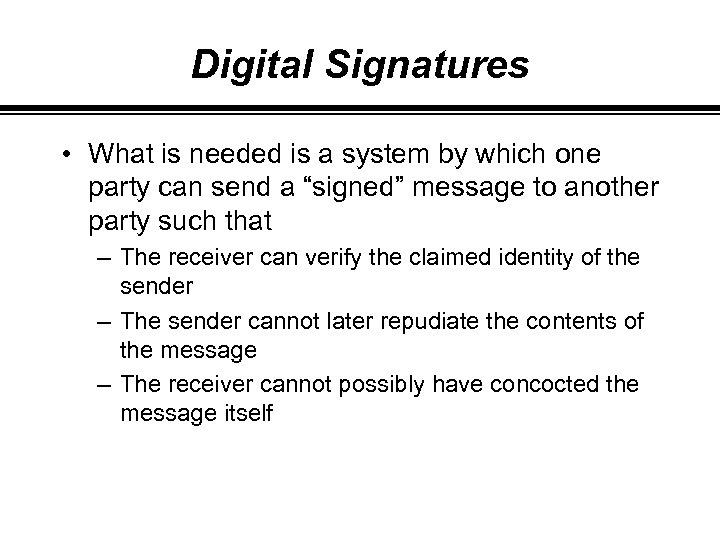Digital Signatures • What is needed is a system by which one party can send a “signed” message to another party such that – The receiver can verify the claimed identity of the sender – The sender cannot later repudiate the contents of the message – The receiver cannot possibly have concocted the message itself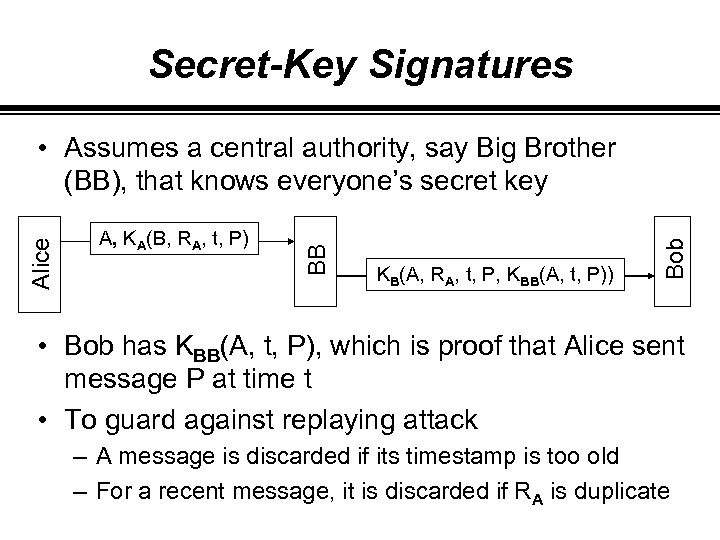Secret-Key Signatures KB(A, RA, t, P, KBB(A, t, P)) Bob A, KA(B, RA, t, P) BB Alice • Assumes a central authority, say Big Brother (BB), that knows everyone’s secret key • Bob has KBB(A, t, P), which is proof that Alice sent message P at time t • To guard against replaying attack – A message is discarded if its timestamp is too old – For a recent message, it is discarded if RA is duplicate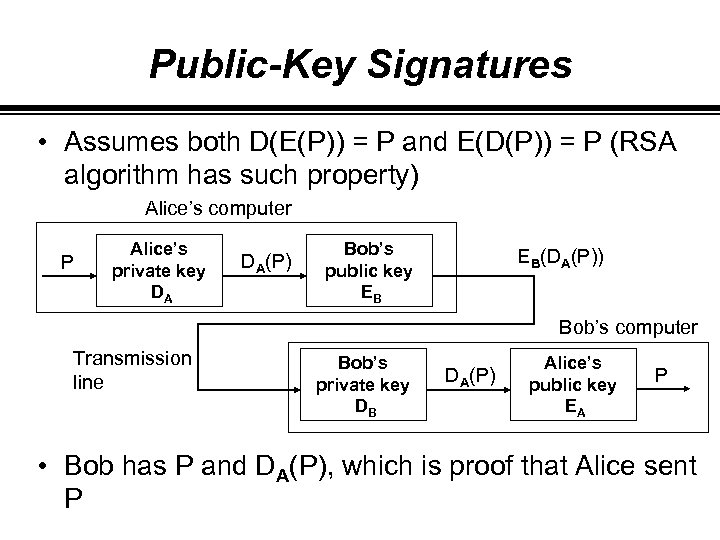Public-Key Signatures • Assumes both D(E(P)) = P and E(D(P)) = P (RSA algorithm has such property) Alice’s computer P Alice’s private key DA DA(P) Bob’s public key EB EB(DA(P)) Bob’s computer Transmission line Bob’s private key DB DA(P) Alice’s public key EA P • Bob has P and DA(P), which is proof that Alice sent P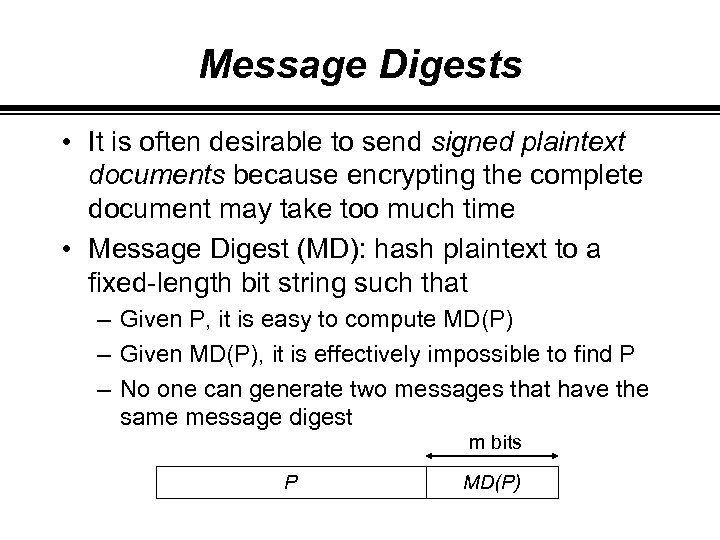Message Digests • It is often desirable to send signed plaintext documents because encrypting the complete document may take too much time • Message Digest (MD): hash plaintext to a fixed-length bit string such that – Given P, it is easy to compute MD(P) – Given MD(P), it is effectively impossible to find P – No one can generate two messages that have the same message digest m bits P MD(P)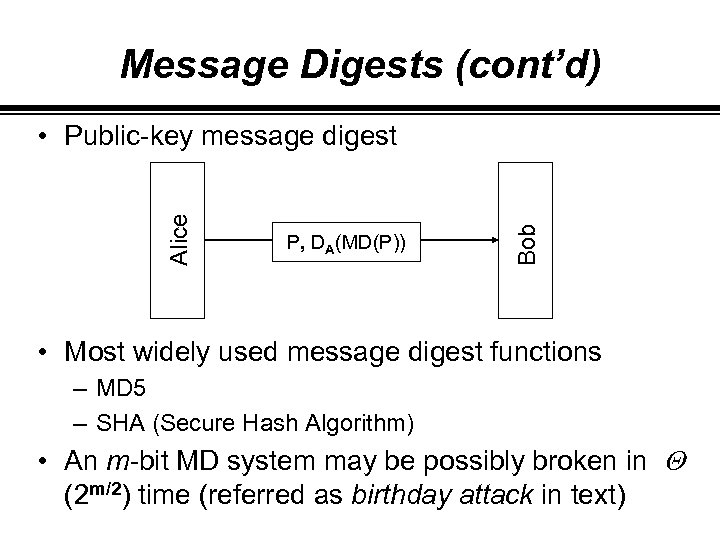Message Digests (cont’d) P, DA(MD(P)) Bob Alice • Public-key message digest • Most widely used message digest functions – MD 5 – SHA (Secure Hash Algorithm) • An m-bit MD system may be possibly broken in Q (2 m/2) time (referred as birthday attack in text)